https://doi.org/10.5194/amt-14-1851-2021
https://doi.org/10.5194/amt-14-1851-2021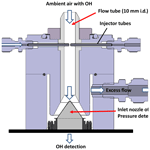# Characterization of a chemical modulation reactor (CMR) for the measurement of atmospheric concentrations of hydroxyl radicals with a laser-induced fluorescence instrument

Changmin Cho, Andreas Hofzumahaus, Hendrik Fuchs, Hans-Peter Dorn, Marvin Glowania, Frank Holland, Franz Rohrer, Vaishali Vardhan, Astrid Kiendler-Scharr, Andreas Wahner, and Anna Novelli
Abstract

Precise and accurate hydroxyl radical (OH) measurements are essential to investigate mechanisms for oxidation and transformation of trace gases and processes leading to the formation of secondary pollutants like ozone (O3) in the troposphere. Laser-induced fluorescence (LIF) is a widely used technique for the measurement of ambient OH radicals and was used for the majority of field campaigns and chamber experiments. Recently, most LIF instruments in use for atmospheric measurements of OH radicals introduced chemical modulation to separate the ambient OH radical concentration from possible interferences by chemically removing ambient OH radicals before they enter the detection cell (Mao et al., 2012; Novelli et al., 2014a). In this study, we describe the application and characterization of a chemical modulation reactor (CMR) applied to the Forschungszentrum Jülich LIF (FZJ-LIF) instrument in use at the atmospheric simulation chamber SAPHIR (Simulation of Atmospheric PHotochemistry In a large Reaction Chamber). Besides dedicated experiments in synthetic air, the new technique was extensively tested during the year-round Jülich Atmospheric Chemistry Project (JULIAC) campaign, in which ambient air was continuously flowed into the SAPHIR chamber. It allowed for performing OH measurement comparisons with differential optical absorption spectroscopy (DOAS) and investigation of interferences in a large variety of chemical and meteorological conditions. Good agreement was obtained in the LIF–DOAS intercomparison within instrumental accuracies (18 % for LIF and 6.5 % for DOAS) which confirms that the new chemical modulation system of the FZJ-LIF instrument is suitable for measurement of interference-free OH concentrations under the conditions of the JULIAC campaign (rural environment). Known interferences from O3+H2O and the nitrate radical (NO3) were quantified with the CMR in synthetic air in the chamber and found to be 3.0×105 and 0.6×105 cm−3, respectively, for typical ambient-air conditions (O3=50 ppbv, H2O = 1% and NO3=10 pptv). The interferences measured in ambient air during the JULIAC campaign in the summer season showed a median diurnal variation with a median maximum value of 0.9×106 cm−3 during daytime and a median minimum value of 0.4×106 cm−3 at night. The highest interference of 2×106 cm−3 occurred in a heat wave from 22 to 29 August, when the air temperature and ozone increased to 40 C and 100 ppbv, respectively. All observed interferences could be fully explained by the known O3+H2O interference, which is routinely corrected in FZJ-LIF measurements when no chemical modulation is applied. No evidence for an unexplained interference was found during the JULIAC campaign.

A chemical model of the CMR was developed and applied to estimate the possible perturbation of the OH transmission and scavenging efficiency by reactive atmospheric trace gases. These can remove OH by gas phase reactions in the CMR or produce OH by non-photolytic reactions, most importantly by the reaction of ambient HO2 with NO. The interfering processes become relevant at high atmospheric OH reactivities. For the conditions of the JULIAC campaign with OH reactivities below 20 s−1, the influence on the determination of ambient OH concentrations was small (on average: 2 %). However, in environments with high OH reactivities, such as in a rain forest or megacity, the expected perturbation in the currently used chemical modulation reactor could be large (more than a factor of 2). Such perturbations need to be carefully investigated and corrected for the proper evaluation of OH concentrations when applying chemical scavenging. This implies that chemical modulation, which was developed to eliminate interferences in ambient OH measurements, itself can be subject to interferences that depend on ambient atmospheric conditions.

Share
Dates
1 Introduction

The hydroxyl radical (OH) plays an important role in tropospheric photochemistry as the main daytime oxidant of trace gases (e.g. volatile organic compounds, VOCs; nitrogen oxides, ${\mathrm{NO}}_{x}=\mathrm{NO}+{\mathrm{NO}}_{\mathrm{2}}$; and carbon monoxide, CO), removing primary pollutants while contributing to ozone (O3) and particle formation (Levy, 1971; Carslaw et al., 2010; Li et al., 2019). In the lower troposphere, OH is mainly produced by two types of atmospheric reactions: first, by solar photolysis of trace gases (most importantly ozone and nitrous acid, HONO),

$\begin{array}{}\text{(R1)}& {\mathrm{O}}_{\mathrm{3}}+h\mathit{\nu }\left(<\mathrm{340}\phantom{\rule{0.125em}{0ex}}\mathrm{nm}\right)\to \text{O}{\left(}^{\mathrm{1}}\text{D}\right)+{\mathrm{O}}_{\mathrm{2}}\text{(R2)}& \text{O}{\left(}^{\mathrm{1}}\text{D}\right)+{\mathrm{H}}_{\mathrm{2}}\mathrm{O}\to \mathrm{2}\mathrm{OH}\text{(R3)}& \mathrm{HONO}+h\mathit{\nu }\left(<\mathrm{400}\phantom{\rule{0.125em}{0ex}}\mathrm{nm}\right)\to \mathrm{OH}+\mathrm{NO},\end{array}$

and second, by reaction of NO with hydroperoxy radicals (HO2),

$\begin{array}{}\text{(R4)}& {\mathrm{HO}}_{\mathrm{2}}+\mathrm{NO}\to \mathrm{OH}+{\mathrm{NO}}_{\mathrm{2}},\end{array}$

which are produced by photolysis of formaldehyde (HCHO) and as intermediates in the OH-initiated degradation of CO and VOCs or the ozonolysis of alkenes. A large number of measurements of OH radical concentrations in ambient air have been conducted in various environments so far (Stone et al., 2012; Feiner et al., 2016; Tan et al., 2017; Mallik et al., 2018; Tan et al., 2018; Whalley et al., 2018; Ma et al., 2019; Lew et al., 2020). Some of these field studies included the detection of peroxy radicals, trace gases and measurements of the OH reactivity (kOH), the inverse of OH lifetime, which helped with investigation of the atmospheric OH radical budget. Good agreement between measured OH radicals and calculations using chemical box modelling that represent the current understanding of tropospheric chemistry is often found at high NO concentrations (>1 parts per billion, ppbv), which favour non-photolytic OH production by Reaction (R4). In pristine environments (NO<1 ppbv), where OH reactivity is dominated by biogenic VOCs, in particular isoprene, a large discrepancy, up to a factor of 10, was found with the measured OH radical concentrations being higher than calculated. This discrepancy suggested that an unknown atmospheric OH formation process is missing from the chemical mechanisms (Tan et al., 2001; Lelieveld et al., 2008; Hofzumahaus et al., 2009; Kubistin et al., 2010; Whalley et al., 2011; Rohrer et al., 2014).

Theoretical (Peeters et al., 2009; da Silva et al., 2010; Peeters and Müller, 2010; Peeters et al., 2014; Wang et al., 2018; Møller et al., 2019) and experimental studies (Crounse et al., 2011; Berndt, 2012; Crounse et al., 2012; Wolfe et al., 2012; Fuchs et al., 2013, 2014; Teng et al., 2017; Fuchs et al., 2018; Berndt et al., 2019; Novelli et al., 2020) have hypothesized and found significant regeneration of OH radicals from unimolecular reactions of organic peroxy radicals (RO2, with R being an organic group) originating from the oxidation of biogenic VOCs such as isoprene, methacrolein and methyl vinyl ketone (MVK). Although the inclusion of these new reaction paths in atmospheric chemical models increases the predicted concentration of OH considerably for some conditions, for example by a factor of 3 over tropical forests (Novelli et al., 2020), it is often not enough to explain the high OH concentrations observed in field experiments in areas characterized by high isoprene emissions (Stone et al., 2011; Lu et al., 2012; Stone et al., 2012; Fuchs et al., 2013; Lu et al., 2013; Wolfe et al., 2014; Feiner et al., 2016; Lew et al., 2020).

An explanation for the remaining discrepancies between measured and modelled OH radical concentrations in high-isoprene and low-NO environments could be interferences in OH radical measurements. In most of the field campaigns described above, the OH radical was measured by gas expansion of ambient air into a low-pressure volume, where the radicals are detected by laser-induced fluorescence (LIF) at 308 nm, except for the study by Wolfe et al. (2014), where the OH radical was measured by a chemical ionization mass spectrometer (CIMS). Laser wavelength modulation is usually applied to distinguish the OH fluorescence from laser-excited background signals. Previous studies have investigated and reported possible interferences in the detection of ambient OH by LIF, which originate from the formation of OH inside the instrument. Such internal OH can be formed by laser photolysis of ozone in the presence of water (Reactions R1 and R2) (Holland et al., 2003; Ren et al., 2004) and acetone (Ren et al., 2004; Fuchs et al., 2016), ozonolysis of alkenes (Ren et al., 2004; Novelli et al., 2014b; Fuchs et al., 2016; Rickly and Stevens, 2018), and unknown reactions of nitrate radicals (NO3) (Fuchs et al., 2016). Most of these interfering species are thought not to play a role at ambient concentrations with one exception. Ozone photolysis in humid air may be relevant but can be corrected for based on laboratory characterization experiments (Holland et al., 2003).

In accordance with a recommendation from the International HOx Workshop 2015 (Hofzumahaus and Heard, 2016), the majority of LIF instruments now apply chemical modulation in order to correct possible interferences. Chemical modulation in instruments measuring OH, which is also commonly used by CIMS, which can measure OH by addition of SO2 in the form of ${\mathrm{HSO}}_{\mathrm{4}}^{-}$ by ionization with ${\mathrm{NO}}_{\mathrm{3}}^{-}$ (Eisele and Tanner, 1991; Tanner et al., 1997; Berresheim et al., 2000; Mauldin et al., 2010), involves periodic scavenging of the ambient OH by addition of a reactant (propane or hexafluoropropene) before the air enters the detection cell. Any remaining OH fluorescence signal can then be attributed to OH produced inside the detection cell (Mao et al., 2012; Novelli et al., 2014a; Tan et al., 2017; Rickly and Stevens, 2018; Tan et al., 2018, 2019; Woodward-Massey et al., 2020) (Table 1). The difference between the signal without and with the scavenger provides interference-free ambient OH signals which are then used to calculate OH radical concentrations by means of a calibration.

Table 1Characteristics of chemical modulation reactors for different OH LIF instruments.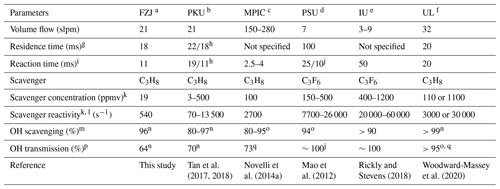a Forschungszentrum Jülich, Germany. b Peking University, Beijing, China. c Max Planck Institute for Chemistry, Mainz, Germany. d Pennsylvania State University, PA, USA. e Indiana University, IN, USA. f University of Leeds, UK. g Total transit time. h The shorter time applies to the CMR version as used in Tan et al. (2018). i Transit time from the injectors to the inlet. j The shorter time was used by Feiner et al. (2016). k Assuming homogeneous mixing in the CMR flow. l The scavenging reactivity was calculated assuming homogeneous mixing. The used rate coefficients for the C3H8 and C3F6+OH radical are $\mathrm{1.1}×{\mathrm{10}}^{-\mathrm{12}}$ and $\mathrm{2.08}×{\mathrm{10}}^{-\mathrm{12}}$ cm3 s−1 at 298 K, respectively (Dubey et al., 1996; Atkinson et al., 2006). m Corresponds to $\left(\mathrm{1}-\mathit{\alpha }\right)×\mathrm{100}$ %. n Measured in synthetic air. o Measured in ambient air with OH produced photolytically by Hg lamp radiation. p Corresponds to ${\mathit{\beta }}_{{\mathrm{N}}_{\mathrm{2}}}×\mathrm{100}$ %. q Measured in ambient air with natural OH at daytime.

Several LIF instruments applying chemical modulation (MPIC, PSU and IU in Table 1) have shown relatively large OH interferences which seem to depend on the chemical conditions of the sampled air as well as on specific instrument characteristics (e.g. inlet size and shape or whether the detection cell is single-path or multiple-path) and/or from the combination of both. After subtraction of the interference, a significantly improved agreement was found between measured OH concentrations and chemical box model predictions (Mao et al., 2012; Feiner et al., 2016; Mallik et al., 2018; Lew et al., 2020). In forest environments, measured interferences contributed 40 %–80 % to the total signal in daytime and 50 %–100 % in nighttime (Mao et al., 2012; Hens et al., 2014; Novelli et al., 2014a; Feiner et al., 2016; Lew et al., 2020). Smaller contributions of 20 %–40 % to the total signal were found in daytime coastal (Mallik et al., 2018), rural (Novelli et al., 2014a) and urban (Brune et al., 2016; Griffith et al., 2016) environments, but nighttime observation were similar to those found in forested environments. Some studies suggest that the interferences may be partly caused by the dissociation of stabilized Criegee intermediates (SCIs) that are produced by ozonolysis of alkenes (Novelli et al., 2014b, 2017; Rickly and Stevens, 2018). However, other LIF instruments using chemical modulation (PKU and Leeds in Table 1) showed insignificant unexplained interferences after the well-quantified photolytic ozone interference had been subtracted (Tan et al., 2017, 2018, 2019; Woodward-Massey et al., 2020). Although these studies were not performed in forested environments with large BVOC (biogenic volatile organic compound) emissions as some of the studies where a large interference was observed, during the summer campaign in Wangdu (Tan et al., 2017) and Beijing (Woodward-Massey et al., 2020), high isoprene concentrations (up to 3 and 7.9 ppbv, respectively) did not seem to perturb the level of the interference. These field campaign results are consistent with the results of laboratory and/or chamber studies (Fuchs et al., 2016; Woodward-Massey et al., 2020) which found insignificant interference from the ozonolysis of BVOCs under atmospheric conditions. An open question remains as to which chemical species and processes produce unexplained OH interferences in some of the LIF instruments and how technical design and operating conditions of the instruments influence the magnitude of the unexplained interferences. Chemical modulation seems to be an appropriate way to minimize such measurement artefacts. However, as will be discussed in this paper, the accurate evaluation of chemical modulation measurements can be challenging and may have systematic errors in the measured OH concentration because chemical reactions of atmospheric trace gases can disturb the efficiency of chemical modulation.

In this study, the chemical modulation reactor (CMR) used in the LIF instrument of Forschungszentrum Jülich (FZJ) is introduced, described and characterized. The characterization includes laboratory tests and chamber experiments. Furthermore, a theoretical model is developed that provides estimates of the possible influence of ambient atmospheric conditions on the chemical modulation efficiency and can possibly be used for the correction of such influences. Measurements of OH radicals using the CMR LIF technique are compared to measurements by differential optical absorption spectroscopy (DOAS) in synthetic and ambient air inside the atmospheric simulation chamber SAPHIR (Simulation of Atmospheric PHotochemistry In a large Reaction Chamber). Most experiments were part of the Jülich Atmospheric Chemistry Project (JULIAC), in which ambient air from 50 m height was continuously drawn through the chamber for 1 month in each season of the year. This allowed for the investigation of possible interference signals for a large range of chemical and meteorological conditions in a rural environment.

2 Methods

## 2.1 Forschungszentrum Jülich LIF OH instrument with chemical modulation reactor (FZJ-LIF-CMR)

The LIF instrument for the measurement of OH, HO2 and RO2 radicals in use at Forschungszentrum Jülich has been extensively described in several previous studies (Holland et al., 2003; Fuchs et al., 2008, 2012a). Briefly, the measurement of OH radicals is achieved by sampling ambient air through a 0.4 mm pinhole nozzle into a low-pressure (4 hPa) detection cell. The OH radical is resonantly excited by a single-path ultraviolet (UV) laser pulse (308 nm) on a single rovibronic transition (Q1(3)) of the ${A}^{\mathrm{2}}{\mathrm{\Sigma }}^{+}-{\mathrm{X}}^{\mathrm{2}}\mathrm{\Pi }$ (0,0) electronic system using a narrow-bandwidth tunable dye laser. The resulting OH fluorescence is detected by a micro-channel plate photomultiplier (MCP) connected to a gated photon counting system. To distinguish the OH fluorescence from non-resonant laser-excited background, the excitation wavelength is modulated on and off the peak of the OH absorption line (Hofzumahaus et al., 1996). The laser system is operated at a high repetition rate of 8.5 kHz, and the MCP signals are integrated over 25 and 10 s in the on- and off-resonance mode, respectively. A complete cycle takes 45 s. The measured OH concentration resulting from the wavelength modulation is hereafter called OHWAVE following the nomenclature introduced by Mao et al. (2012).

$\begin{array}{}\text{(1)}& {\left[\mathrm{OH}\right]}_{\mathrm{WAVE}}=\frac{{S}_{\mathrm{WAVE}}}{{C}_{\mathrm{OH}}}=\frac{\mathrm{1}}{{C}_{\mathrm{OH}}}×\left({S}_{\mathrm{on}}-{S}_{\mathrm{off}}\right)\end{array}$

Here, SWAVE is the OH radical fluorescence signal normalized to the UV laser power. It is obtained from the difference between Son and Soff, the on- and off-resonance signals, respectively. COH represents the OH radical detection sensitivity, which is obtained during the calibration of the instrument. The instrument is calibrated for OH radicals by illuminating humidified synthetic air (Linde, N2 and O2 purity >99.99990 %) by a mercury lamp thereby producing a known amount of OH radicals (approximately 5.0×109 cm−3 at 0.8 % water vapour mixing ratio) by photolysis of water vapour at 185 nm. The intensity of the light is determined by actinometry, and it is measured by a calibrated photo-diode. The 1σ accuracy of the calibration is 10 % (Holland et al., 2003). The detailed design of the radical source and the calibration procedure are described in previous studies (Holland et al., 2003; Fuchs et al., 2011).

Hydroxyl radicals originating not from ambient air but formed within the detection cell will also be detected if they are excited by the laser in the detection volume. To quantify the interference signal from internally generated OH radicals, a chemical modulation reactor (CMR) has been developed and mounted in front of the OH detection cell of both the LIF system permanently mounted at the SAPHIR chamber (in this study) and the LIF system used in field campaigns (Tan et al., 2017, 2018, 2019). The CMR outline is shown in Fig. 1, and instrumental characteristics are listed in Table 1. The reactor consists of a 79 mm long PTFE (polytetrafluoroethylene) Teflon tube with 10 mm internal diameter that is mounted in an aluminium body. Two stainless-steel injectors ($\mathrm{1}/\mathrm{8}$ in. o.d. tubes with 50 µm i.d.) are located 50 mm above the nozzle pinhole pointing to the centre of the CMR tube. For typical measurement conditions, 20.6 slpm (1 slpm =1 L min−1 at 1 atm and 20 C) of ambient air is sampled. A constant flow of 500 sccm (1 sccm =1 cm3 min−1 at 1 atm and 20 C) of nitrogen (N2, purity >99.9990 %) or a mixture of nitrogen and the OH scavenger (Air Liquide, propane, purity >99.95 %; 5.0±0.1 % mixture in nitrogen, purity >99.999 %) regulated by mass flow controllers is injected into the main stream. When propane is added, the resulting propane mixing ratio downstream of the injectors is typically 19 ppmv. The concentration of propane was selected to scavenge OH radicals efficiently (90 %–95 %) in the CMR but to avoid scavenging OH radicals in the detection cell of the instrument (see Sect. 3.2). Propane is used as an OH scavenger because it does not photolyse at the wavelength of the laser and does not react with other oxidants (e.g. O3) which could produce OH radicals in the CMR. When switching from the mixture of a scavenger and nitrogen to pure nitrogen, the injection lines are flushed for 15 s at a higher flow rate of 700 sccm to remove any residual scavenger molecules from the lines. The measurement time with addition of propane is 135 s, followed by another period of 135 s without propane injection. A complete cycle therefore takes 270 s. The flow rate behind the injectors is 21.1 slpm. It is controlled by a flow controller (Bronkhorst, low Δ−p) connected to a membrane pump which removes 20 slpm of excess air while the OH measurement cell samples 1.1 slpm.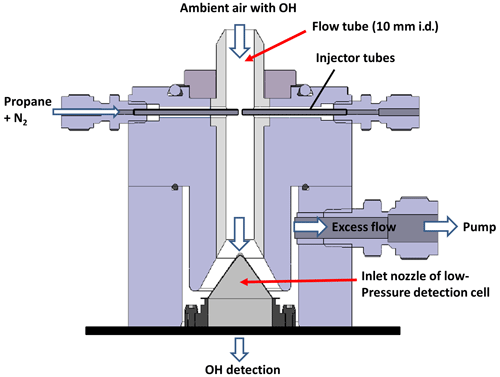Figure 1Schematic drawing of the chemical modulation reactor (CMR) in front of the OH LIF detection cell. Ambient air is drawn through a PFA (perfluoroalkoxy alkane) flow tube (79 mm length; 10 mm inner diameter). A flow of 1.1 slpm is expanded through an 0.4 mm inlet nozzle into a low-pressure cell for OH detection, and 20 slpm of excess air are removed by a pump. A nitrogen flow of 0.5 slpm containing 19 ppmv propane as an OH scavenger is added via two $\mathrm{1}/\mathrm{8}$ in. (o.d.) injector tubes (50 µm i.d.) 50 mm above the nozzle. The distance between the injector tips is less than 1 mm. All flows (nitrogen flow, excess flow and nozzle flow) are held constant during operation.

Without added scavenger (N2 mode of operation), the OH signal obtained from an on- and off-resonance measurement cycle is

$\begin{array}{}\text{(2)}& {S}_{\mathrm{OH}}^{{\mathrm{N}}_{\mathrm{2}}}={S}_{\mathrm{OH}}+{S}_{\mathrm{i}}.\end{array}$

It contains the signal SOH from ambient OH radicals which pass the CMR and reach the inlet nozzle of the detection cell and potentially an interference signal Si from OH radicals that are produced inside the cell (internal OH). When the scavenger is added (sc mode of operation), a large percentage (typically 96 %) of atmospheric OH radicals are removed by reaction with propane. The OH signal in the scavenger mode is

$\begin{array}{}\text{(3)}& {S}_{\mathrm{OH}}^{\mathrm{sc}}=\mathit{\alpha }{S}_{\mathrm{OH}}+{S}_{\mathrm{i}}.\end{array}$

It consists of the OH signal from the residual ambient OH, which is reduced by a factor α due to scavenging, and Si. The residual factor α has to be determined experimentally (see Sect. 3), in order to calculate SOH and Si from a set of measurements in the scavenging and nitrogen modes.

$\begin{array}{}\text{(4)}& {S}_{\mathrm{OH}}=\frac{{S}_{\mathrm{OH}}^{{\mathrm{N}}_{\mathrm{2}}}-{S}_{\mathrm{OH}}^{\mathrm{sc}}}{\mathrm{1}-\mathit{\alpha }}\text{(5)}& {S}_{\mathrm{i}}=\frac{{S}_{\mathrm{OH}}^{\mathrm{sc}}-\mathit{\alpha }}{{S}_{\mathrm{OH}}^{{\mathrm{N}}_{\mathrm{2}}}}\mathrm{1}-\mathit{\alpha }\end{array}$

The conversion of SOH into an interference-free ambient OH concentration, called [OH]CHEM, requires calibration.

$\begin{array}{}\text{(6)}& \left[\mathrm{OH}{\right]}_{\mathrm{CHEM}}=\frac{\mathrm{1}}{{C}_{\mathrm{OH}}{\mathit{\beta }}_{{\mathrm{N}}_{\mathrm{2}}}}{S}_{\mathrm{OH}}\end{array}$

In addition to the detection sensitivity COH of the OH detection cell without the CMR, the OH transmission of the CMR in the N2 mode (${\mathit{\beta }}_{{\mathrm{N}}_{\mathrm{2}}}$) needs to be known. In the present system, the transmission is reduced due to wall loss reactions and has a typical value of 64 %. The quantities COH and ${\mathit{\beta }}_{{\mathrm{N}}_{\mathrm{2}}}$ have to be calibrated either separately or together (${C}_{\mathrm{OH}}×{\mathit{\beta }}_{{\mathrm{N}}_{\mathrm{2}}}$) by calibrating the OH cell with the CMR in place (see Sect. 3).

The interference OH signal Si can be converted into an equivalent ambient OH concentration in analogy to Eq. (6).

$\begin{array}{}\text{(7)}& \left[\mathrm{OH}{\right]}_{\mathrm{i}}=\frac{\mathrm{1}}{{C}_{\mathrm{OH}}{\mathit{\beta }}_{{\mathrm{N}}_{\mathrm{2}}}}{S}_{\mathrm{i}}\end{array}$

It should be noted that the application of the CMR relies on the assumption that interfering chemical species, which produce OH inside the instrument, are not affected by switching between N2 and scavenger injection in the CMR. Furthermore, it is assumed that internal OH is not scavenged by propane inside the OH detection cell. The latter assumption was confirmed for this instrument (see Sect. 3).

A large suite of additional instruments was available for experimental studies in the SAPHIR chamber. Measurements of interest are listed in Table 2 with 1σ accuracies and precisions. The calibration-free differential optical absorption spectroscopy (DOAS) instrument (White cell, absorption path length: 2.2 km) provided an absolute reference for OH radical measurements with a 1σ accuracy of 6.5 % (Hausmann et al., 1997; Schlosser et al., 2007, 2009). The pressure and temperature dependence of the OH absorption cross section has been discussed in detail in the study by Dorn et al. (1995). Within the natural variance of the atmospheric pressure and a temperature interval of ±20 K around room temperature, the OH cross section changes less than 2 %. The LIF instrument for OH concentration measurement includes two more measurement cells for the detection of HO2 and RO2 radicals. In the second LIF cell (HOx cell), HO2 is indirectly measured by chemical conversion to OH by NO (Linde, mixture of 1 % NO in nitrogen (purity >99.999 %)) (Fuchs et al., 2011). During the JULIAC experiments, NO (2.5×1013 cm−3) was used for minimizing a possible interference from specific RO2 radicals as suggested in Fuchs et al. (2011). OH reactivity (kOH) was measured by a laser-photolysis laser-induced fluorescence instrument (LP-LIF) (Lou et al., 2010; Fuchs et al., 2017b). Photolysis frequencies were calculated from spectral actinic flux densities measured by a spectroradiometer (Bohn et al., 2005; Bohn and Zilken, 2005). In addition, NO3 radical concentrations were monitored by a custom-built cavity ring-down spectroscopy (FZJ-CRDS) instrument; nitrous acid (HONO) was monitored by a long-path absorption photometer (LOPAP) (Kleffmann et al., 2006; Li et al., 2014); the water vapour mixing ratio was monitored by a cavity ring-down spectroscopy instrument (CRDS, Picarro); nitric oxide (NO) and nitrogen dioxide (NO2) were monitored by a chemiluminescence (CL) instrument with a photolytic converter (ECO PHYSICS); and O3 was monitored by UV absorption instruments (Ansyco 41M and Thermo Scientific 49i) that agree within 5 %.

Table 2Specification of instruments used at SAPHIR in this study.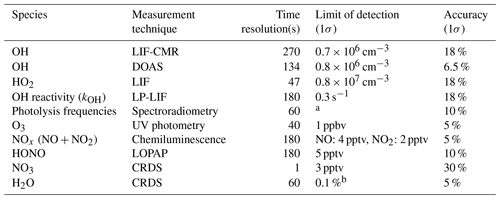a Several orders of magnitude lower than the maximum value at noon. b Volume mixing ratio.

## 2.3 Atmospheric simulation chamber SAPHIR

Comprehensive tests of the FZJ-LIF-CMR instrument with synthetic (Sect. 3) and ambient air (Sect. 4) were performed in the atmosphere simulation chamber SAPHIR on the campus of Forschungszentrum Jülich, Germany, as the instrument is permanently mounted in the SAPHIR chamber. A detailed description of the chamber has been presented in previous publications (Bohn and Zilken, 2005; Rohrer et al., 2005; Fuchs et al., 2010b). Briefly, the chamber has a cylindrical shape (length: 18 m, diameter: 5 m and volume: 270 m3) and is made of a double Teflon (FEP; fluorinated ethylene propylene) film, which has a light transmission greater than 0.8 over the complete solar spectral range (Bohn and Zilken, 2005). A slight overpressure is maintained inside the chamber, and clean nitrogen is used to flush the volume between the inner and outer FEP films to prevent contamination from outside the chamber. Two fans are mounted inside the chamber to ensure a homogeneous mixing of trace gases.

The SAPHIR chamber is an ideal tool to test and characterize instruments for the measurement of atmospheric trace gases, as was shown for the measurements of OH (Schlosser et al., 2007, 2009; Fuchs et al., 2012a), HO2 (Fuchs et al., 2010b), HO2 (Fuchs et al., 2010a), NO3 (Dorn et al., 2013), N2O5 (Fuchs et al., 2012b) and OH reactivity (Fuchs et al., 2017a). In this study, the chamber was used for experiments with synthetic air (Linde, purity: > 99.99990 %) to test the CMR for known interferences from ozone and water and NO3 radicals (Sect. 3) and with ambient air (Sect. 4).

## 2.4 JULIAC campaign

The CMR system was further used for OH measurements in SAPHIR within the Jülich Atmospheric Chemistry Project (JULIAC) in 2019, during which ambient air was continuously flowed through the chamber. The campus is surrounded by a mixed deciduous forest and is located close to the small town of Jülich. Therefore, ambient air is expected to be influenced by both anthropogenic and biogenic emissions. The JULIAC campaign consisted of four intensive phases, each carried out within one season (Table 3). It was designed to examine the seasonal and diurnal cycle of atmospheric oxidants in a mixed environment and the role of oxidants in the degradation of VOCs, NOx, and formation and ageing of particles. Ambient air was sampled at 50 m height through a SilcoNert® coated inlet line (104 mm i.d.) at a flow rate of 660 m3 h−1. Around 250 m3 h−1 of this airflow was directed towards the SAPHIR chamber. This new inlet system for SAPHIR enables all instruments to measure the same air composition without the perturbation of steady-state conditions by local emitters. The inlet line was gently heated (+1 to 2 C above ambient temperature) to avoid water vapour condensation in the line. A cyclone was positioned upstream of the SAPHIR chamber to remove particles with a diameter larger than 10 µm. The residence time of the ambient air in the chamber was about 1 h, giving enough time for radicals and short-lived trace gases to reach a new steady state and smoothing atmospheric variability. Daytime-averaged meteorological conditions and trace gas concentrations during each JULIAC intensive phase are summarized in Table 3, and nighttime-averaged data are shown in Table S2 in the Supplement. Daytime is defined by the condition that the HO2 photolysis frequency is greater than $\mathrm{0.5}×{\mathrm{10}}^{-\mathrm{4}}$ s−1. In Table 3 and S2, the total OH reactivity of non-methane VOCs (kVOC) is calculated by subtracting the contribution of CO, CH4, O3, NO and NO2 from the measured OH reactivity. The tables show that a large range of chemical and meteorological conditions were encountered during the four different JULIAC campaigns, allowing for the investigation of possible interferences in the OH radical measurement for different scenarios (e.g. summer and winter, high and low NO and O3, and high and low humidity) in a rural environment.

Table 3Summary of meteorological conditions and trace gas concentrations during JULIAC given as daytime median values with 1σ standard deviations of ambient variabilities.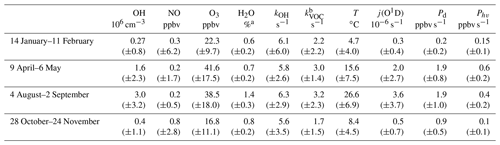a Volume mixing ratio. b OH reactivity of non-methane VOCs, calculated as the difference between measured total kOH and the sum of calculated reactivities of CH4, CO, O3, NO and NO2.

3 Characterization and test of the CMR for clean-air conditions

This section describes the characterization and test of the CMR for conditions where the measured air is very clean. This condition was simulated by performing experiments in synthetic air.

## 3.1 CMR transmission

The loss of OH radicals in the CMR and the scavenging efficiency were determined in laboratory experiments using the photolytic radical source which is also used for absolute calibration of the instrument. As is usual, OH radicals are produced by 185 nm photolysis of water vapour in a flow of synthetic air at 1 atm and room temperature, where the concentration of formed OH is determined from the humidity, volume flow and the intensity of the 185 nm radiation. The latter is observed by a phototube which can be traced back to a measurement of ozone which is produced in the same gas flow by 185 nm photolysis of oxygen (for details see Fuchs et al., 2011). The radical source was operated with a total flow of 24 slpm, which overflowed the inlet of the CMR tube. The length of the laminar flow tube (18.7 mm i.d. with a frit at the top and a Reynolds number Re of 1920) of the radical source is 20 cm, resulting in plug flow condition that ensures a uniform distribution of OH and O3 in the calibration gas. This condition was confirmed by ozone measurements with a modified chemiluminescence instrument (50 pptv limit of detection), showing that the ozone concentration in the central flow was the same within a few percent compared to the mean ozone concentration leaving the radical source.

Several tests were performed to characterize heterogeneous OH losses at surfaces in the CMR. Three reactive regions can be distinguished (Fig. 2): the entrance section above the injectors, the injector tubes and the reaction section downstream of the injectors. The flow in the entrance section is in the transition regime between laminar and turbulent (Reynolds number Re=2800), but turbulence is further increased by the injectors which protrude approximately 4 mm into the flow tube. In order to quantify potential OH loss on the surface of the injectors, the stainless-steel injectors were replaced by Teflon tubes of the same geometry for a test. Within measurement precision (±3 %), no difference in the detected OH was found when the material of the injectors was changed. Since Teflon and stainless steel have surface reaction probabilities for radicals which are different by orders of magnitude (Rozhenshtein et al., 1985), the result suggests that OH loss at the injector surfaces is negligible regardless of its material.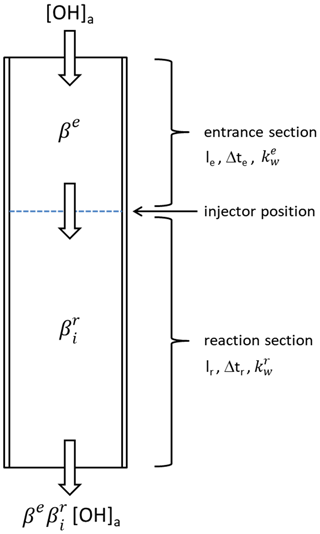Figure 2Schematic of the chemical modulation reactor (CMR). The flow tube has an entrance section with a length le up to the injector position. In this section, OH radicals are lost by wall reaction during the transit time Δte with a rate coefficient ${k}_{\mathrm{w}}^{\mathrm{e}}$, resulting in an OH transmission βe. Behind the injector position follows the reaction zone, where OH is scavenged when propane is injected into the flow. The reaction zone has a length lr with a transit time Δtr and wall loss rate coefficient ${k}_{\mathrm{w}}^{\mathrm{r}}$. The OH transmission ${\mathit{\beta }}_{\mathrm{i}}^{\mathrm{r}}$ in the reaction section depends on the chemical modulation mode (i =N2 when pure nitrogen is injected and i = sc when a scavenger–nitrogen mixture is injected). The total transmission for OH is given by the product ${\mathit{\beta }}^{\mathrm{e}}{\mathit{\beta }}_{\mathrm{i}}^{\mathrm{r}}$.

Since the injector tubes do not cause OH losses, the OH transmission in the N2 mode (${\mathit{\beta }}_{{\mathrm{N}}_{\mathrm{2}}}$) can be described as the product of transmissions of the entrance section (βe) and reaction section (${\mathit{\beta }}_{{\mathrm{N}}_{\mathrm{2}}}^{\mathrm{r}}$) (see Fig. 2).

$\begin{array}{}\text{(8)}& {\mathit{\beta }}_{{N}_{\mathrm{2}}}={\mathit{\beta }}^{\mathrm{e}}×{\mathit{\beta }}_{{N}_{\mathrm{2}}}^{\mathrm{r}}\end{array}$

As a next step, the values of βe and ${\mathit{\beta }}_{{\mathrm{N}}_{\mathrm{2}}}^{\mathrm{r}}$ were determined experimentally using clean air as a carrier gas for OH. The clean-air condition (kOH=0) will be indicated by the superscript 0 in the following. First, the OH transmission of the CMR tube without built-in injectors was determined by measuring OH from the radical source with and without the CMR mounted on the OH cell. A transmission ${\mathit{\beta }}_{\mathrm{tube}}^{\mathrm{0}}$ of 0.81±0.02 was determined for a flow rate of 21.1 slpm. Assuming that the OH loss is caused by only wall reactions and follows first-order kinetics, a rate coefficient kw=11.8 s−1 is calculated from

$\begin{array}{}\text{(9)}& {\mathit{\beta }}_{\mathrm{tube}}^{\mathrm{0}}=\mathrm{exp}\left(-{k}_{\mathrm{w}}\mathrm{\Delta }t\right),\end{array}$

where Δt is the transit time through the tube. The rate coefficient kw that was obtained can be used to estimate the transmission of the entrance section (βe,0),

$\begin{array}{}\text{(10)}& {\mathit{\beta }}^{\mathrm{e},\mathrm{0}}=\mathrm{exp}\left(-{k}_{\mathrm{w}}^{\mathrm{e}}\mathrm{\Delta }{t}_{\mathrm{e}}\right),\end{array}$

which has a transit time Δte=6.6 ms, yielding ${\mathit{\beta }}^{\mathrm{e},\mathrm{0}}=\mathrm{0.92}$.

With built-in $\mathrm{1}/\mathrm{8}$ in. injectors and nitrogen injection, an OH transmission of ${\mathit{\beta }}_{{\mathrm{N}}_{\mathrm{2}}}^{\mathrm{0}}=\mathrm{0.64}±\mathrm{0.03}$ was measured for the total CMR. The wall loss of OH radicals for a flow rate in the CMR between 12 and 21.1 slpm was investigated (Fig. S1). When the flow rate increases, it has two opposing effects. First, it decreases the residence time in the CMR, leading to a steady increase of the OH transmission from about 60 % at 12 slpm to 65 % at 15 slpm. Second, an increasing flow rate causes more turbulence and faster radial transport to the wall. This is the likely reason for flattening of the transmission curve at flow rates above 15 slpm. A further increase of the flow rate of more than 21 slpm does not improve the OH transmission in synthetic air. To minimize the possible effect of secondary chemistry, the CMR was operated with the fastest flow rate achievable (21.1 slpm), which corresponds to a transmission a transmission ${\mathit{\beta }}_{{\mathrm{N}}_{\mathrm{2}}}^{\mathrm{0}}$ of 0.64.

Using the experimental values of ${\mathit{\beta }}_{{\mathrm{N}}_{\mathrm{2}}}^{\mathrm{0}}$ and βe,0, the relationship (Eq. 8) yields ${\mathit{\beta }}_{{\mathrm{N}}_{\mathrm{2}}}^{\mathrm{r},\mathrm{0}}=\mathrm{0.69}$ as the transmission of the reaction section. With a transit time Δtr=11.2 ms, a wall loss rate coefficient ${k}_{\mathrm{w}}^{\mathrm{r}}$ of 33 s−1 is obtained.

The same procedure was used to determine also the CMR transmission for HO2 radicals, which provides information needed to calculate OH production in the CMR by Reaction R4 (Sect. 4). In this case, the calibration source was operated with added CO (300 ppmv) in order to convert all OH to HO2 in the calibration gas (Fuchs et al., 2011). In order to detect HO2, the CMR was mounted on a HOx detection cell. The measured transmissions and wall loss rate coefficients for HO2 are listed in Table 4 together with the results for OH.

Table 4Transmission and wall loss rate coefficients for OH and HO2 in the chemical modulation reactor ($\mathrm{1}/\mathrm{8}$ in. injectors) when nitrogen is injected.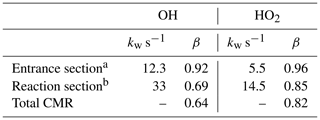a Transit time is 6.6 ms. b Transit time is 11.2 ms.

The rate coefficients for HO2 are a factor of 2 smaller than for OH because HO2 is less reactive. If the wall loss was limited by the reactive collision frequency at the wall surfaces, a larger difference would be expected because the reaction probability γ on Teflon surfaces is about a factor of 10 smaller for HO2 compared to OH (Rozhenshtein et al., 1985). The rate coefficient ks for wall loss due to reactive surface collisions can be calculated for a cylindrical tube with radius R by

$\begin{array}{}\text{(11)}& {k}_{\mathrm{s}}=\mathit{\gamma }\frac{\stackrel{\mathrm{‾}}{u}}{\mathrm{2}R}\end{array}$

if transport in the gas phase presents no resistance (Zasypkin et al., 1997). For OH with a mean molecular velocity $\stackrel{\mathrm{‾}}{u}$ of 610 m s−1 (298 K) and γ of $\mathrm{2.5}×{\mathrm{10}}^{-\mathrm{3}}$ for Teflon surfaces (Rozhenshtein et al., 1985), the value of ks is 152 s−1. The measured rate coefficients for OH loss (kw) (Table 4) are an order of magnitude smaller, indicating that OH loss in the CMR tube is limited by turbulent transport to the walls, which is about 3 times faster in the reaction section compared to the CMR entrance region.

## 3.2 Scavenging efficiency

The same radical source operated with clean synthetic air was also used for the characterization test of scavenging efficiency. When propane is injected into the CMR, the chemistry in the reaction section changes. When no propane is injected (N2 mode), OH is only lost by wall reaction.

$\begin{array}{}\text{(12)}& {\mathit{\beta }}_{{N}_{\mathrm{2}}}^{\mathrm{r},\mathrm{0}}=\mathrm{exp}\left(-{k}_{\mathrm{w}}^{\mathrm{r}}\mathrm{\Delta }{t}_{\mathrm{r}}\right)\end{array}$

In the scavenger mode, additional OH loss occurs by the gas phase reaction with propane. The OH transmission is then given by

$\begin{array}{}\text{(13)}& {\mathit{\beta }}_{\mathrm{sc}}^{\mathrm{r},\mathrm{0}}=\mathrm{exp}\left(-\left[{k}_{\mathrm{w}}^{\mathrm{r}}+{k}_{\mathrm{sc}}\right]\mathrm{\Delta }{t}_{\mathrm{r}}\right),\end{array}$

where ksc is the pseudo-first-order rate constant for a reaction between OH and propane.

$\begin{array}{}\text{(14)}& {k}_{\mathrm{sc}}={k}_{\mathrm{OH}+\mathrm{propane}}\left[\mathrm{propane}\right]\end{array}$

Here, kOH+propane is the bimolecular rate constant for scavenging, which has a value of $\mathrm{1.1}×{\mathrm{10}}^{-\mathrm{12}}$ cm3 s−1 at 298 K (Atkinson et al., 2006).

The fraction of ambient OH remaining that subsequently enters the measurement cell downstream of the CMR when the CMR is operated in the scavenging mode is the ratio of ${\mathit{\beta }}_{\mathrm{sc}}^{\mathrm{r}}$ to ${\mathit{\beta }}_{{\mathrm{N}}_{\mathrm{2}}}^{\mathrm{r}}$.

$\begin{array}{}\text{(15)}& \mathit{\alpha }=\frac{{\mathit{\beta }}_{\mathrm{sc}}^{\mathrm{r}}}{{\mathit{\beta }}_{{\mathrm{N}}_{\mathrm{2}}}^{\mathrm{r}}},\end{array}$

which in the specific case of synthetic air (kOH=0) becomes

$\begin{array}{}\text{(16)}& {\mathit{\alpha }}^{\mathrm{0}}=\frac{{\mathit{\beta }}_{\mathrm{sc}}^{\mathrm{r},\mathrm{0}}}{{\mathit{\beta }}_{{\mathrm{N}}_{\mathrm{2}}}^{\mathrm{r},\mathrm{0}}}=\mathrm{exp}\left(-{k}_{\mathrm{sc}}\mathrm{\Delta }{t}_{\mathrm{r}}\right),\end{array}$

where α0 was experimentally determined for OH in synthetic air from the radical source by calculating the ratio of OH signals measured with and without any scavenger. The measured ratio is denoted α0, where the superscript indicates that the sampled air does not contain any additional OH reactants (kOH=0). Note that the value of the residual factor α is different for ambient air (kOH≠0) as is discussed in Sect. 4. Figure 3a and b show the measured dependence of α0 on the added amount of propane for different instrumental conditions. For small amounts of propane, α0 decreases nearly exponentially as expected from Eq. (16), whose derivation assumes homogenous mixing of the scavenger. At higher scavenger concentrations, however, α0 levels off into a weak dependency and decreases more slowly than expected from an exponential decay. This behaviour indicates inhomogeneous mixing of propane in the CMR flow.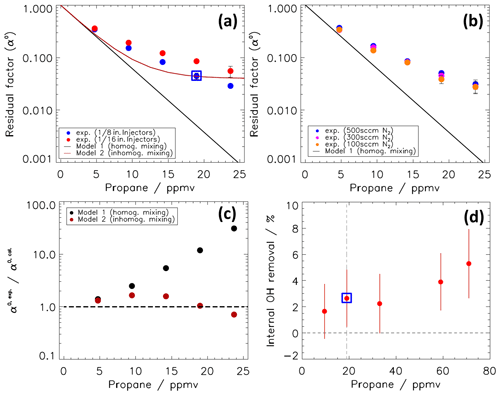Figure 3(a) Residual factor (α0) for OH in a flow of humidified synthetic air running through the CMR. The OH air mixture is provided by a photolytic OH radical source. α0 is shown as a function of the calculated concentration of propane (at 298 K and 1 atm), which is injected as a scavenger with 500 sccm N2 into the CMR flow. The experimental α0 values are shown for two types of injectors with different outer diameters ($\mathrm{1}/\mathrm{8}$ and $\mathrm{1}/\mathrm{16}$ in.). The blue open squares indicate the condition used for normal operation in this work. Measurements are compared to calculations assuming either homogeneous or inhomogeneous mixing. (b) Same as (a), showing measured α0 values for different injector flow rates. (c) Ratio of experimental and modelled α0 for the case with $\mathrm{1}/\mathrm{8}$ in. injectors and 500 sccm N2 injector flow. (d) Measured OH removal by internal scavenging inside the OH detection cell (see text).

Figure 3a also shows that the use of $\mathrm{1}/\mathrm{8}$ in. injectors leads to improved mixing compared to $\mathrm{1}/\mathrm{16}$ in. injectors. The likely reason is that the $\mathrm{1}/\mathrm{8}$ in. injectors produce a larger flow resistance within the CMR and produce higher turbulence in the flow because of their larger surface. The disadvantage of the $\mathrm{1}/\mathrm{8}$ in. injectors is a smaller CMR transmission, ${\mathit{\beta }}_{{\mathrm{N}}_{\mathrm{2}}}^{\mathrm{0}}=\mathrm{0.64}$, compared to a value of 0.75 for the $\mathrm{1}/\mathrm{16}$ in. injectors. Thus, the faster mixing of propane using the $\mathrm{1}/\mathrm{8}$ in. injectors goes along with a higher OH wall loss.

Figure 3b shows that the carrier gas (N2) flow rate has only a minor influence on α0, when the injector flow rate is changed between 100 and 500 sccm. In order to ensure fast exchange of the gases in the injector lines, the higher flow rate of 500 sccm was chosen for routine operation. For the majority of the results shown in this study, the $\mathrm{1}/\mathrm{8}$ in. injectors were used to maximize the mixing in the CMR. The $\mathrm{1}/\mathrm{16}$ in. injectors were only tested during part of the JULIAC experiments (Table S1).

Ideally, α would be zero. In that case, the OH signal in the scavenger mode would be identical to the interference signal (${S}_{\mathrm{i}}={S}_{\mathrm{OH}}^{\mathrm{sc}}$), and the ambient OH signal would simply be given by the signal difference of both modes (${S}_{\mathrm{OH}}={S}_{\mathrm{OH}}^{{\mathrm{N}}_{\mathrm{2}}}-{S}_{\mathrm{OH}}^{\mathrm{sc}}$). Higher propane concentrations should result in smaller α values, but Fig. 3a shows that a further increase of the propane concentration beyond 20 ppmv gives only small improvements in α. In order to avoid OH scavenging in the detection cell by propane concentrations that are too high that would lead to an underestimation of the interference signal, the propane concentration in the CMR was chosen as 19 ppmv for routine operation. Note that 19 ppmv would be the nominal value if the injected propane is homogeneously mixed into the total CMR flow. For this condition, 96 % of OH is scavenged (α0=0.042). This value corresponds to an effective scavenging rate coefficient ${k}_{\mathrm{sc}}^{\mathrm{eff}}$ of 283 s−1 in Eq. (16). It is a factor of 1.8 smaller than calculated for complete homogenous mixing (ksc=513 s−1).

A plausible explanation for the weaker-than-expected dependence of α on the propane concentration is incomplete mixing of the injected scavenger. A minor fraction f of a few percent of the total CMR flow containing little or no scavenger would explain the observed dependence of α0 in Fig. 3a. The fraction with no scavenger ($k{{}^{\prime }}_{\mathrm{sc}}=\mathrm{0}$) can be imagined as gas filaments with ${\mathit{\alpha }}_{\mathrm{1}}\left(k{{}^{\prime }}_{\mathrm{sc}}=\mathrm{0}\right)$ of 1 embedded in the turbulent flow. The bulk flow, i.e. the major part (1−f), would contain all the injected propane well mixed, with ${\mathit{\alpha }}_{\mathrm{2}}\left(k{{}^{\prime \prime }}_{\mathrm{sc}}={k}_{\mathrm{OH}+\mathrm{propane}}\left[\mathrm{Propane}\right]\right)$. The resulting α0 of the total CMR flow would then be

$\begin{array}{}\text{(17)}& \begin{array}{rl}{\mathit{\alpha }}^{\mathrm{0}}& \phantom{\rule{0.25em}{0ex}}=\left(\mathrm{1}-f\right){\mathit{\alpha }}_{\mathrm{2}}\left(k{{}^{\prime \prime }}_{\mathrm{sc}}\right)+f{\mathit{\alpha }}_{\mathrm{1}}\left(k{{}^{\prime }}_{\mathrm{sc}}=\mathrm{0}\right)\\ & \phantom{\rule{0.25em}{0ex}}=\left(\mathrm{1}-f\right){\mathit{\alpha }}_{\mathrm{2}}\left(k{{}^{\prime \prime }}_{\mathrm{sc}}\right)+f.\end{array}\end{array}$

Using Eqs. (16) and (17), we obtain

$\begin{array}{}\text{(18)}& {\mathit{\alpha }}^{\mathrm{0}}=\left(\mathrm{1}-f\right)\mathrm{exp}\left(k{{}^{\prime \prime }}_{\mathrm{sc}}\mathrm{\Delta }{t}_{\mathrm{r}}\right)+f.\end{array}$

This is a simple model for the case of inhomogeneous mixing with an assumed value of f=0.04, which provides a better description of the observed dependence of α on the propane concentration than Eq. (16) (Fig. 3a and c). In this model, the calculated reactivity $k{{}^{\prime \prime }}_{\mathrm{sc}}$ is 534 s−1, which is larger than the ksc for complete homogeneous mixing, as the injected propane is assumed to be mixed in a smaller volume flow (96 % of the total volume flow).

## 3.3 Scavenging of internal OH

As mentioned above, the concentration of propane is chosen not to scavenge any OH radicals in the detection cell. Calculations suggest that less than 1 % of OH radicals are scavenged in the detection cell at 19 ppmv of propane because of the low pressure (4 hPa) and the short residence time (3 ms) in the detection cell. A laboratory test to quantify internal OH removal in the detection cell was proposed by Woodward-Massey et al. (2020) and was also done in this study. As employed in the HO2 transmission tests, the radical source was operated as a pure HO2 source. The CMR was mounted on the HOx detection cell, which used an NO concentration 10 times higher than during typical operation for ambient HO2 concentration measurements by an injection of 8 sccm of 10 % NO (Linde NO 10 %, mixture in nitrogen) to achieve a high HO2-to-OH radical conversion efficiency of 85 %. The HO2 radical concentration provided by the radical source is not affected by the propane injection in the CMR, but the HO2 is converted to OH in the detection cell. If a significant number of OH radicals were scavenged by propane inside the detection cell, a difference between the measured signals with and without propane injection in the CMR would be observed. Different propane concentrations (10 to 75 ppmv) were tested during the experiment (Fig. 3d). A small amount of (3±2) % of internal OH was scavenged when 19 ppmv of propane was applied. An increase of internal removal up to a value of (5±3) % was observed for the highest propane mixing ratio (75 ppmv). The internal-scavenging value of (3±2) % for the operation conditions in this work applies to OH radicals, which are internally formed and exposed to reaction with propane on a similar timescale as in the HO2-to-OH conversion (ca. 2 ms) in the test experiment. OH that is produced photolytically in the laser beam has a much shorter residence time (ca. 0.1 ms) before it is removed by the fast gas flow and will therefore be much less affected by internal scavenging. As discussed by Woodward-Massey et al. (2020), internal OH that is immediately formed after the sampled air has passed the inlet nozzle would have a longer residence time, which could be larger by up to a factor of 2 compared to the HO2 conversion experiment. Even in this case, the internal scavenging would have a small effect and is therefore considered negligible.

## 3.4 OH detection sensitivity and calibration

As mentioned in Sect. 2.1, the conversion of interference-free ambient OH signals SOH into ambient concentrations requires the experimental determination of the parameters COH and ${\mathit{\beta }}_{{\mathrm{N}}_{\mathrm{2}}}$ (Eq. 6). The combined detection sensitivity ${C}_{\mathrm{OH}}×{\mathit{\beta }}_{{\mathrm{N}}_{\mathrm{2}}}^{\mathrm{0}}$ of the measurement system with the mounted CMR was calibrated with the radical source, which provided a known OH concentration in humidified synthetic air. The superscript 0 in ${\mathit{\beta }}_{{\mathrm{N}}_{\mathrm{2}}}^{\mathrm{0}}$ indicates that the calibration was done in synthetic air (kOH=0). The calibration was independently tested by an OH measurement comparison against the OH DOAS instrument which provides an absolute reference for OH concentrations in the SAPHIR chamber. The chamber was filled with clean synthetic air. Only water vapour (1.3 %) was injected, and the chamber roof was opened, allowing for the photolytic formation of OH radicals from HONO, which is photochemically produced at the chamber walls (Rohrer et al., 2005). Figure 4 shows the OH radical concentrations measured by DOAS and LIF-CMR ([OH]CHEM). The difference between the measured time series is on average $\left(\mathrm{0.29}±\mathrm{0.9}\right)×{\mathrm{10}}^{\mathrm{6}}$ cm−3, which is less than 5 % of the average measured OH concentrations between 08:30 and 12:00. Thus, the instruments agreed well within the combined 1σ accuracies of the LIF-CMR calibration (±18 %) and DOAS (±6.5%), which confirms the LIF calibration. A linear regression (not shown) which considers the precision of both instruments using the “fitexy” algorithm (Press, 1992) between LIF-CMR and DOAS yields a slope of 0.97. The good agreement gives confidence in the applied chemical modulation technique under the operational conditions and in the laboratory-determined parameters of α0 and ${C}_{\mathrm{OH}}×{\mathit{\beta }}_{{\mathrm{N}}_{\mathrm{2}}}^{\mathrm{0}}$ used for data evaluation.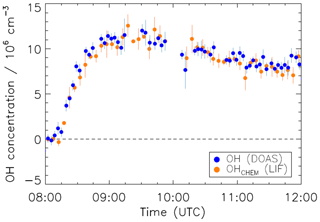Figure 4Time series of OH concentrations measured by DOAS and LIF (with CMR) in humidified synthetic air in the SAPHIR chamber. The measured OH reactivity in the chamber air was less than 2 s−1. The chamber was illuminated by solar radiation, and OH was mainly produced by solar photolysis of traces of nitrous acid emitted from the chamber walls. The data shown are averaged over 300 s.

The implementation of chemical modulation in the FZJ-LIF instrument has specific disadvantages compared to the operation of the LIF OH detection without the CMR. It requires a longer measurement time because half of the time is spent for the measurement of interferences in the scavenger mode. In the current work, measurement of ambient OH (N2 mode, 135 s) contains three on- and off-resonance cycles which yield three ambient OH data points (45 s). The subsequent scavenger mode (135 s) again contains three on- and off-resonance cycles. Another disadvantage of the CMR is the reduction of the OH detection sensitivity by a factor of 1.6 due to wall loss in the CMR, yielding a 1σ limit of detection (LOD) of 7×105 cm−3 (Table 2). Note that this LOD value applies to a single OH data point of 45 s duration.

Accuracy and precision of the OH measurements are generally worsened because [OH]CHEM requires more experimental parameters (COH, α and ${\mathit{\beta }}_{{\mathrm{N}}_{\mathrm{2}}}$) for evaluation than OHWAVE, as well as more measurement modes, each of which contribute additional noise. The 1σ accuracy of OH measurements depends on the error of the radical source (10 %) and the reproducibility of the experimental determination of ${C}_{\mathrm{OH}}×{\mathit{\beta }}_{{\mathrm{N}}_{\mathrm{2}}}^{\mathrm{0}}$ and α0. During the 1-year JULIAC campaign (Sect. 4), the reproducibilities were 15 % and 10 %, respectively, resulting in a total 1σ accuracy of ±18 %. Additional uncertainties arise when air pollutants influence the chemistry in the CMR (see Sect. 4).

## 3.5 Interference tests

The main purpose of the CMR is to discriminate the signal of ambient OH radicals from signals of known and unknown interferences. Two types of known interferences (from ozone photolysis and NO3 radicals) were re-investigated in this work by making use of the chemical modulation technique. These tests were done in synthetic air to avoid potential interferences from other sources.

### 3.5.1 Interference from ozone photolysis

It is well-known that internal OH can be produced in the 308 nm laser beam of LIF systems by photolysis of O3 in humid air according to Reactions (R1) and (R2) (Holland et al., 2003).

The interference is proportional to the concentrations of ozone and water vapour and laser power.

In the past, OHWAVE measurements by the FZJ-LIF instrument were routinely corrected for this interference based on laboratory characterization. Similar tests were repeated here without and with the CMR for a range of conditions (Fig. 5). Mixing ratios of ozone and water vapour were varied up to 450 ppbv and 1.8 %, respectively. Laser power was modulated between 10 and 20 mW. A laboratory test was performed with the calibration source as a gas supply with a total flow of 11 slpm and the photolysis lamp switched off. Ozone was added by a home-built ozone generator and measured in the excess air with UV photometry (Ansyco). Relative humidity was monitored by a humidity sensor (HMT333, Vaisala). In two additional experiments in the SAPHIR chamber, water vapour and ozone were added in synthetic air in dark conditions (chamber roof closed), and their concentrations were measured by the instruments listed in Table 2. The OH interferences observed in the laboratory and SAPHIR experiments scale linearly with the product of ozone and water vapour mixing ratios as expected (Eq. 19 and Fig. 5). All three experiments with and without the CMR agree with each other within 15 %. On average, the interference is equivalent to an OH concentration of $\left(\mathrm{3.4}±\mathrm{0.3}\right)×{\mathrm{10}}^{\mathrm{5}}$ cm−3 per 50 ppbv of O3 and 1 % water mixing ratio, which is at the limit of detection for the LIF instrument without the CMR, and a factor of 2 below the limit of detection with the CMR. The value of the interference agrees well with earlier determinations for the FZJ-LIF instrument with reported values of $\left(\mathrm{2.7}±\mathrm{0.8}\right)×{\mathrm{10}}^{\mathrm{5}}$ cm−3 (Holland et al., 1998) and $\left(\mathrm{3.2}±\mathrm{0.8}\right)×{\mathrm{10}}^{\mathrm{5}}$ cm−3 (Holland et al., 2003) for the same conditions.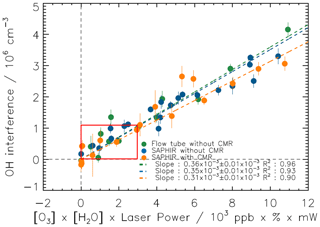Figure 5OH interference, expressed as equivalent ambient OH concentration, from the laser photolysis of ozone in humidified synthetic air for a range of concentrations of ozone, water vapour and laser power. Tests were done with and without the CMR with a flow tube or in chamber experiments. Measurements were fitted to a linear function forced through the origin. The red box specifies the interference for concentrations of ozone and water vapour normally observed in the lower troposphere.

### 3.5.2 Interference from NO3

Fuchs et al. (2016) have reported an OH interference from NO3 radicals by an unknown mechanism producing OH in the FZJ-LIF instrument. The observed interference was independent of water vapour in the gas phase and independent of laser power. The reported interference signal in the presence of 10 pptv NO3 was equivalent to an atmospheric OH concentration of 1.1×105 cm−3. In the present work, the interference from NO3 was re-determined applying chemical modulation. The experiments were performed in the SAPHIR chamber using thermal decomposition of N2O5 added from a condensed source which produces NO3 radicals. Figure 6 shows a linear increase of the measured OH interference with increasing NO3 concentration, which is measured by a cavity ring-down instrument similar to the instrument described in Fuchs et al. (2009) and Wagner et al. (2011). A linear regression analysis yields a value that is equivalent to an OH concentration of 5.8×104 cm−3 per 10 pptv NO3, which is negligible for typical ambient concentrations of NO3. The value is roughly 2 times smaller than the result of Fuchs et al. (2016). The reason for the discrepancy between the two tests is unclear. One reason could be an additional NO3 heterogeneous loss to the wall of the CMR, like HO2 and OH loss, when the CMR is applied. As the origin of the NO3 radical interference in our LIF instrument is unknown; it cannot be ruled out that other experimental conditions affected the interfering signal. In any case, the small interference from NO3 is negligible for OH measurements for the FZJ-LIF instrument, regardless of whether chemical modulation is used or not. A similar conclusion was drawn from experiments by Woodward-Massey et al. (2020) for the LIF instrument used by the University of Leeds (UK).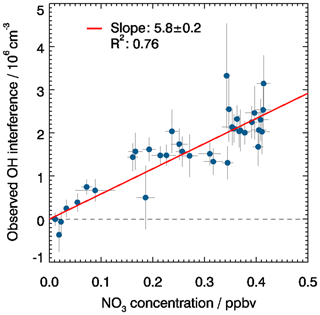Figure 6Correlation between the measured OH interference, determined by chemical modulation, and the NO3 concentration measured during an experiment when NO3 (from thermal decomposition of frozen N2O5 crystals) was present in the chamber. Measurements were fitted to a linear function forced through the origin.

4 Chemical modulation measurements in ambient air

This section describes the application of chemical modulation in a real atmosphere with numerous atmospheric pollutants. Section 4.1 analyses the potential influence of atmospheric chemical conditions on the performance of the chemical modulation system, and Sect. 4.2 shows test results from the JULIAC field campaign.

## 4.1 Sensitivity study of the influence of ambient-air conditions on chemical modulation

### 4.1.1 Kinetic model

When polluted air with sufficiently high OH reactivity (kOH) is sampled by the CMR, the reaction of OH with atmospheric reactants competes with the OH loss by wall reactions and scavenging inside the CMR. Therefore, an influence of kOH on the OH transmission and scavenging efficiency is expected. In addition, some reactions that produce OH in the ambient air continue when the air enters the CMR. A simple kinetic model is used here to quantify these influences.

In the atmosphere, the ambient concentration of short-lived OH radicals is in a steady state and can be calculated from the total OH production rate and OH reactivity.

$\begin{array}{}\text{(20)}& \left[\mathrm{OH}{\right]}_{\mathrm{a}}=\frac{{P}_{hv}+{P}_{\mathrm{d}}}{{k}_{\mathrm{OH}}}\end{array}$

Here, Phv represents the photolytic OH production rate, which is dominated in the lower troposphere by the photolysis of O3 and HONO (Reactions R1–R3). Pd denotes non-photolytic (dark) OH production, which includes the reaction of HO2 radicals with NO (Reaction R4), ozonolysis of alkenes and, for example, isomerization reactions of RO2 leading directly to OH formation.

When atmospheric OH radicals enter the inner darkness of the chemical modulation reactor, photolysis will stop, but chemical reactions of OH with atmospheric reactants and dark OH production will continue, as long as the reactants that produce or destroy OH are not lost in the CMR tube. In many atmospheric environments, the reaction between HO2 and NO (Reaction R4) with a reaction rate constant of ${k}_{{\mathrm{HO}}_{\mathrm{2}}+\mathrm{NO}}=\mathrm{8.1}×{\mathrm{10}}^{-\mathrm{12}}$ cm3 s−1 at 298 K (Atkinson et al., 2004) is the dominant non-photolytic source of atmospheric OH. It can be assumed that the OH production rate from Reaction (R4) continues inside the CMR at the same rate as in the ambient atmosphere because concentrations of HO2 and NO do not significantly change. HO2 is relatively short-lived, but during the short transit time of 18 ms through the reactor, its conversion to OH is small for typical conditions. Even high ambient NO mixing ratios of 30ppbv lead only to a conversion of HO2 to OH of less than 10 %. Furthermore, HO2 loss will be partly compensated by HO2 production from the reaction of atmospheric RO2 with NO. Also, the loss of HO2 by wall reactions is small (18 %) (Sect. 3.1). NO has an even longer chemical lifetime than HO2, which is usually determined by the reaction of NO with O3 and peroxy radicals (HO2 and RO2).

As a result of the ongoing chemical reactions, ambient OH will relax in the CMR to a new steady state with concentration [OH] and chemical lifetime $\mathit{\tau }{{}^{\prime }}_{\mathrm{OH}}$.

$\begin{array}{}\text{(21)}& \left[\mathrm{OH}\right]{}^{\prime }=\frac{{P}_{\mathrm{d}}}{{k}_{\mathrm{OH}}+{k}_{\mathrm{w}}+{k}_{\mathrm{sc}}}\text{(22)}& \mathit{\tau }{{}^{\prime }}_{\mathrm{OH}}=\frac{\mathrm{1}}{{k}_{\mathrm{OH}}+{k}_{\mathrm{w}}+{k}_{\mathrm{sc}}}\end{array}$

The relaxation follows first-order kinetics, and the reaction time t in the reactor determines the degree of relaxation. The general time law for such a transition from an initial concentration [OH]0 (t=0) to a final concentration [OH] is given by

$\begin{array}{}\text{(23)}& \begin{array}{rl}& \left[\mathrm{OH}\right]\left(t\right)\\ & \phantom{\rule{0.25em}{0ex}}\phantom{\rule{0.25em}{0ex}}\phantom{\rule{0.25em}{0ex}}={\left[\mathrm{OH}\right]}_{\mathrm{0}}+\left\{\left[\mathrm{OH}\right]{}^{\prime }-{\left[\mathrm{OH}\right]}_{\mathrm{0}}\right\}\left[\mathrm{1}-\mathrm{exp}\left(-\frac{t}{\mathit{\tau }{{}^{\prime }}_{\mathrm{OH}}}\right)\right].\end{array}\end{array}$

Using this expression, an OH transmission β can be defined for a tube section with transit time Δt.

$\begin{array}{}\text{(24)}& \mathit{\beta }=\frac{\left[\mathrm{OH}\right]\left(\mathrm{\Delta }t\right)}{{\left[\mathrm{OH}\right]}_{\mathrm{0}}}\end{array}$

The initial concentration ([OH]0) can be expressed as a reduced ambient OH concentration c[OH]a, with a reduction factor c of 1.0 for the entrance section and c of βe for the reaction section (cf. Fig. 2). Making use of the relationships in Eqs. (20), (21) and (22), the transmission can be generally written as

$\begin{array}{}\text{(25)}& \begin{array}{rl}& \mathit{\beta }\left(c,{k}_{\mathrm{OH}},{k}_{\mathrm{w}},{k}_{\mathrm{sc}},\mathrm{\Delta }t\right)\\ & \phantom{\rule{0.25em}{0ex}}\phantom{\rule{0.25em}{0ex}}\phantom{\rule{0.25em}{0ex}}\phantom{\rule{0.25em}{0ex}}=\mathrm{1}+\left\{\frac{\mathrm{1}}{c}\frac{{P}_{\mathrm{d}}}{{P}_{hv}+{P}_{\mathrm{d}}}×\frac{{k}_{\mathrm{OH}}}{{k}_{\mathrm{OH}}+{k}_{\mathrm{w}}+{k}_{\mathrm{sc}}}-\mathrm{1}\right\}\\ & \phantom{\rule{0.25em}{0ex}}\phantom{\rule{0.25em}{0ex}}\phantom{\rule{0.25em}{0ex}}\phantom{\rule{0.25em}{0ex}}\phantom{\rule{0.25em}{0ex}}\left[\mathrm{1}-\mathrm{exp}\left(-\left[{k}_{\mathrm{OH}}+{k}_{\mathrm{w}}+{k}_{\mathrm{sc}}\right]\mathrm{\Delta }t\right)\right].\end{array}\end{array}$

More specifically, we obtain for the entrance and reaction sections in the N2 and scavenger mode the following transmissions:

$\begin{array}{}\text{(26)}& {\mathit{\beta }}^{\mathrm{e}}=\mathit{\beta }\left(c=\mathrm{1},{k}_{\mathrm{OH}},{k}_{\mathrm{w}}^{\mathrm{e}}{k}_{\mathrm{sc}}=\mathrm{0},\mathrm{\Delta }{t}_{\mathrm{e}}\right),\text{(27)}& {\mathit{\beta }}_{{\mathrm{N}}_{\mathrm{2}}}^{\mathrm{r}}=\mathit{\beta }\left(c={\mathit{\beta }}^{\mathrm{e}},{k}_{\mathrm{OH}},{k}_{\mathrm{w}}^{\mathrm{r}},{k}_{\mathrm{sc}}=\mathrm{0},\mathrm{\Delta }{t}_{\mathrm{r}}\right),\text{(28)}& {\mathit{\beta }}_{\mathrm{sc}}^{\mathrm{r}}=\mathit{\beta }\left(c={\mathit{\beta }}^{\mathrm{e}},{k}_{\mathrm{OH}},{k}_{\mathrm{w}}^{\mathrm{r}},{k}_{\mathrm{sc}},\mathrm{\Delta }{t}_{\mathrm{r}}\right).\end{array}$

These formulas can be inserted in Eqs. (8) and (15) to calculate ${\mathit{\beta }}_{{\mathrm{N}}_{\mathrm{2}}}$ and α as a function of kOH, respectively. Note that for the case of kOH=0, Eqs. (26)–(28) become identical with Eqs. (10), (12) and (13), respectively.

### 4.1.2 Scavenging model for the case of homogeneous mixing

The kinetic model from Sect. 4.1 is used to estimate the influence of the ambient OH reactivity and dark OH production on the determination of interferences and ambient OH concentrations. The OH wall loss rate coefficients, ${k}_{\mathrm{w}}^{\mathrm{e}}$ and ${k}_{\mathrm{w}}^{\mathrm{r}}$, are taken from Table 4. The reaction times are Δte=6.6 ms and Δtr=11.2 ms. We assume homogeneous mixing of the propane in the scavenging mode applying an effective rate coefficient ${k}_{\mathrm{sc}}^{\mathrm{eff}}$ of 283 s−1 that was calculated from the experimental α0 of 0.042 (Sect. 3.1).

As explained in Sect. 2.1, the extraction of SOH and Si from the measured OH signals in the scavenging and nitrogen modes (Eqs. 4 and 5) requires the knowledge of the remaining fraction α of ambient OH in the scavenging mode. Figure 7a presents modelled α as a function of atmospheric OH reactivity for different ratios of dark-to-total OH production rates ${P}_{\mathrm{d}}/\left({P}_{hv}+{P}_{\mathrm{d}}$). The dependence is shown for reactivities from 0 to 100 s−1, a range that is typical for the lower troposphere (e.g. Lou et al., 2010), and for ${P}_{\mathrm{d}}/\left({P}_{hv}+{P}_{\mathrm{d}}$) ratios between 0 and 1. The parameter α shows a considerable variation for these conditions with the largest effect at high OH reactivities. For kOH=100 s−1, α varies from 0.042 to 0.3 for variation of ${P}_{\mathrm{d}}/\left({P}_{hv}+{P}_{\mathrm{d}}$) from 0 to 1. The latter case with PdPhv is typical at nighttime. In the special case of Pd=0, when no OH is produced inside the CMR, the residual factor α remains constant (same as the experimental α0=0.042) and becomes independent of kOH. In this case, kOH adds to the total first-order loss rates and cancels out in Eq. (15), yielding Eq. (16). Low Pd values would be expected, for example, in a low-NO environment, where the reaction of HO2 with NO does not play a significant role. Under atmospheric conditions when the production Pd exceeds the photolytic OH production, the remaining fraction of ambient OH reaching the nozzle increases due to the ongoing dark OH production in the CMR. The sensitivity to kOH increases with ${P}_{\mathrm{d}}/\left({P}_{hv}+{P}_{\mathrm{d}}$) and becomes largest at nighttime, when ambient OH production is controlled by dark reactions only.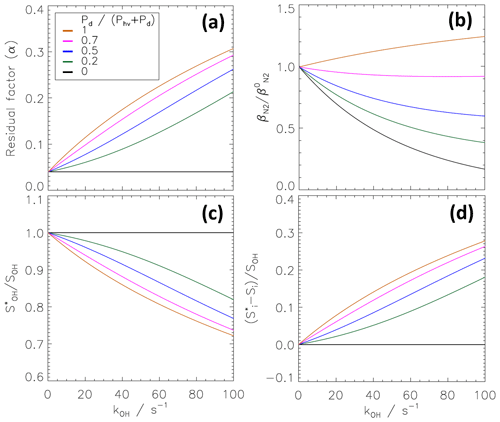Figure 7Theoretical kOH dependence of the residual factor α (a) and normalized transmission ${\mathit{\beta }}_{{\mathrm{N}}_{\mathrm{2}}}/{\mathit{\beta }}_{{\mathrm{N}}_{\mathrm{2}}}^{\mathrm{0}}$ (b). The applied model (Sect. 4.2) assumes homogeneous mixing. Parameters are taken from the characterization experiments of the CMR. The dependence is shown for different ratios of dark to total atmospheric OH production rates. A ratio of one corresponds to nighttime (without photolytic OH production), while a ratio of zero denotes a case without dark OH production. Panels (c, d) show the bias in the determination of the ambient OH signal (SOH) and interference signal (Si), if the kOH dependence of α is not taken into account. ${S}_{\mathrm{OH}}^{*}$ and ${S}_{i}^{*}$ are calculated with α0 instead of α (kOH).

The sensitivity of the calculated signals SOH (Eq. 4) and Si (Eq. 5) to variations of α (Fig. 7a) can be seen in Fig. 7c and d, respectively. A biased signal ${S}_{\mathrm{OH}}^{*}$ would be obtained if the influence of kOH on the chemical modulation was neglected.

$\begin{array}{}\text{(29)}& \frac{{S}_{\mathrm{OH}}^{*}}{{S}_{\mathrm{OH}}}=\frac{\mathrm{1}-\mathit{\alpha }}{\mathrm{1}-{\mathit{\alpha }}^{\mathrm{0}}}\end{array}$

The corresponding biased interference signal ${S}_{\mathrm{i}}^{*}$ is similarly

$\begin{array}{}\text{(30)}& \frac{{S}_{\mathrm{i}}^{*}-{S}_{\mathrm{i}}}{{S}_{\mathrm{OH}}}=\mathrm{1}-\frac{{S}_{\mathrm{OH}}^{*}}{{S}_{\mathrm{OH}}}.\end{array}$

Figure 7d demonstrates that the interference signal would be systematically overdetermined, while the ambient OH signal would be correspondingly underestimated (Fig. 7c). This effect is largest at night, where up to 25 % of the true ambient OH signal would be wrongly assigned as interference at kOH=100 s−1. The error remains generally small (<5 %) for ${P}_{\mathrm{d}}/\left({P}_{hv}+{P}_{\mathrm{d}}\right)$ ratios in the range between 0 and 1, if OH reactivities stay below 10 s−1. More generally, the influence of kOH on α is small when kOH is much lower than the scavenger reactivity (here 283 s−1) and therefore introduces only a small perturbation in the CMR chemistry.

### 4.1.3 Scavenging model for the case of inhomogeneous mixing

The model results in Fig. 7a, c and d assume homogeneous mixing of the scavenger with an effective reactivity of ${k}_{\mathrm{sc}}^{\mathrm{eff}}=\mathrm{283}$ s−1. As an alternative model, Eq. (17) can be used to simulate inhomogeneous mixing. As explained in Sect. 3.2, this model assumes that a small fraction f of 0.04 of air contains little or no scavenger and therefore does not contribute to chemical modulation (α1=1). The main bulk of the gas flow, i.e. part (1−f) of the CMR flow, is assumed to contain the injected propane well mixed with a scavenger reactivity of $k{{}^{\prime \prime }}_{\mathrm{sc}}=\mathrm{534}$ s−1. Equations (16), (27) and (28) are then applied to calculate the kOH dependence of α2 in Eq. (17) to determine α. The result presented in Fig. 8a is very similar to the homogeneous mixing case shown in Fig. 7a. Again, the residual factor α is constant (0.042) and independent of kOH for Pd=0. In this model, the constant offset represents ambient OH that was not scavenged in the minor fraction of air with no scavenger. In the bulk flow, however, essentially all OH is depleted by the high scavenger reactivity of 534 s−1. For Pd>0, α increases with kOH as in the case of homogeneous mixing. The maximum sensitivity to kOH is again obtained for a ${P}_{\mathrm{d}}/\left({P}_{hv}+{P}_{\mathrm{d}}\right)$ ratio of 1. The main difference between the results for the homogeneous and inhomogeneous mixing is a slightly weaker sensitivity to kOH in the case of inhomogeneous mixing due to the higher ksc value in the bulk flow. Thus, α varies from 0.042 to 0.22 at kOH=100 s−1 for inhomogeneous mixing, whereas the span is slightly larger, reaching 0.3, in the homogeneously mixed case. As the results for α are very similar, also the ratio ${S}_{\mathrm{OH}}^{*}/{S}_{\mathrm{OH}}$ gives comparable results (Figs. 7c and 8b).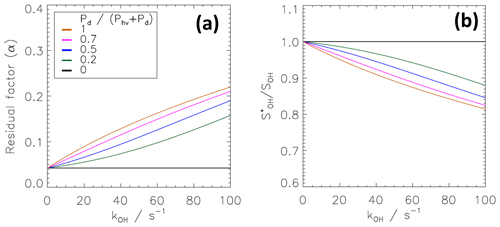Figure 8Calculated kOH dependence of the residual factor α and ratio ${S}_{\mathrm{OH}}^{*}/{S}_{\mathrm{OH}}$ using a model that takes inhomogeneous mixing of the scavenger into account (see Sect. 4.3). The dependence is shown for different ratios of dark to total atmospheric OH production rates. The coloured horizontal lines denote the same ratios of dark to total OH production rates as used in Fig. 7.

### 4.1.4 Transmission model

The conversion of the signals SOH and Si to concentrations requires the knowledge of ${\mathit{\beta }}_{{\mathrm{N}}_{\mathrm{2}}}$ (Eqs. 6 and 7). As the calibration of our LIF-CMR instrument determines ${C}_{\mathrm{OH}}×{\mathit{\beta }}_{{\mathrm{N}}_{\mathrm{2}}}^{\mathrm{0}}$ (Sect. 3.4), Eq. (6) can be rewritten as

$\begin{array}{}\text{(31)}& \left[\mathrm{OH}{\right]}_{\mathrm{CHEM}}=\frac{\mathrm{1}}{{C}_{\mathrm{OH}}{\mathit{\beta }}_{{\mathrm{N}}_{\mathrm{2}}}^{\mathrm{0}}}×\frac{{\mathit{\beta }}_{{\mathrm{N}}_{\mathrm{2}}}^{\mathrm{0}}}{{\mathit{\beta }}_{{\mathrm{N}}_{\mathrm{2}}}}×{S}_{\mathrm{OH}}.\end{array}$

Here, the normalized function ${\mathit{\beta }}_{{\mathrm{N}}_{\mathrm{2}}}/{\mathit{\beta }}_{{\mathrm{N}}_{\mathrm{2}}}^{\mathrm{0}}$ contains the kOH dependence, which is shown in Fig. 7b. ${\mathit{\beta }}_{{\mathrm{N}}_{\mathrm{2}}}/{\mathit{\beta }}_{{\mathrm{N}}_{\mathrm{2}}}^{\mathrm{0}}$ is much more sensitive to OH reactivity from OH reactants contained in the ambient air than the remaining fraction of OH in the scavenger mode α. Without any scavenger, the atmospheric OH reactivity competes only with wall loss, for which the rate is a magnitude similar to kOH. In an environment with little NO, i.e. for ${P}_{\mathrm{d}}/\left({P}_{hv}+{P}_{\mathrm{d}}\right)=\mathrm{0}$, kOH has to be added to the wall loss rate. The normalized ratio ${\mathit{\beta }}_{{\mathrm{N}}_{\mathrm{2}}}/{\mathit{\beta }}_{{\mathrm{N}}_{\mathrm{2}}}^{\mathrm{0}}$ decreases exponentially with $-{k}_{\mathrm{OH}}\left(\mathrm{\Delta }{t}_{\mathrm{e}}+\mathrm{\Delta }{t}_{\mathrm{r}}\right)$ in this case. Under this condition, the normalized transmission decreases by a factor of 6 when kOH reaches 100 s−1. Without taking this effect into account, the calculated ambient OH concentration would be extremely underestimated in an environment with very little NO. However, when the OH reactivity remains below 10 s−1, OH would be underestimated by less than 15 %.

When the value of the ${P}_{\mathrm{d}}/\left({P}_{hv}+{P}_{\mathrm{d}}\right)$ ratio exceeds 0.7, the kOH dependence of the normalized transmission becomes weak. In this case, the normalized transmission lies around 1.0. It exceeds 100 % transmission at ${P}_{\mathrm{d}}/\left({P}_{hv}+{P}_{\mathrm{d}}\right)=\mathrm{1}$. This means that the loss of OH in ambient air during the transit in the CMR is smaller than expected from measurements in clean synthetic air. The condition ${P}_{\mathrm{d}}/\left({P}_{hv}+{P}_{\mathrm{d}}\right)=\mathrm{1}$ implies that the total atmospheric OH production continues when the air enters the CMR. Inside the CMR, OH wall reactions compete with gas phase reactions (kOH). When kOH is much larger than kw, the expected steady state of OH in the CMR is the same value as in outside air, which means that the transmission ${\mathit{\beta }}_{{\mathrm{N}}_{\mathrm{2}}}$ becomes effectively 1.0 and that ${\mathit{\beta }}_{{\mathrm{N}}_{\mathrm{2}}}/{\mathit{\beta }}_{{\mathrm{N}}_{\mathrm{2}}}^{\mathrm{0}}=\mathrm{1}/{\mathit{\beta }}_{{\mathrm{N}}_{\mathrm{2}}}^{\mathrm{0}}$.

## 4.2 Atmospheric OH radical measurements with the FZJ-LIF-CMR during the JULIAC campaign

The JULIAC campaign was designed to investigate tropospheric chemistry in a rural environment which is influenced by biogenic and anthropogenic emissions. The 1-year campaign made use of the SAPHIR infrastructure and instrumentation (Sect. 2.4). During the four intensive JULIAC periods (Tables 3 and S3) OH measurements were performed by LIF with chemical modulation. In addition, OH was measured by DOAS for some of the JULIAC periods (winter (I), summer (III) and autumn (IV) seasons). Besides studying atmospheric chemical processes, the campaign afforded the opportunity to test the chemical modulation technique under natural atmospheric conditions. The CMR was partly operated with different types of injectors and at different propane concentrations (Table S1). For a short time between 1 and 11 February 2019, OH detection was done without the CMR to increase the OH detection sensitivity for measurement of low wintertime OH concentrations.

### 4.2.1 Influence of ambient conditions

The possible influence of ambient conditions on the chemical modulation results was investigated for the conditions of the JULIAC campaign. The ratio ${P}_{\mathrm{d}}/\left({P}_{hv}+{P}_{\mathrm{d}}\right)$ of the dark-to-total OH production was calculated from Reaction (R4) (Pd) and the photolysis of O3 and HONO (Phv). The ratio was between 0.7 and 1.0 most of the time during the spring (II), summer (III) and autumn (IV) of 2019 (Fig. 9). Only in the winter season (I), the range of values was broader (0.4–1.0). The minima of the ratios are due to high NOx concentrations in the morning, which suppresses the HO2 concentration and the OH production by Reaction (R4) until NOx has decreased later in the day. During all seasons, measured OH reactivities were relatively low (<15 s−1) (Table 3) with few exceptions in the winter period where kOH reached 30 s−1. For ambient conditions of JULIAC, the predicted influence of kOH on the parameters α and ${\mathit{\beta }}_{{\mathrm{N}}_{\mathrm{2}}}$ remains generally small (Fig. 10). The influence on the calculated ambient OH signal is mostly less than 5 % during the spring (II), summer (III) and autumn (IV) seasons and not higher than 12 % in the winter season (I) (Fig. 10c). For the encountered ${P}_{\mathrm{d}}/\left({P}_{hv}+{P}_{\mathrm{d}}$) ratios of 0.7–1.0, the normalized transmission ${\mathit{\beta }}_{{\mathrm{N}}_{\mathrm{2}}}/{\mathit{\beta }}_{{\mathrm{N}}_{\mathrm{2}}}^{\mathrm{0}}$ is only weakly dependent on kOH, and the variability is less than ±5 % in most cases (Fig. 10b). When ambient OH concentrations are calculated (Eqs. 4 and 6), the influences of kOH on α and ${\mathit{\beta }}_{{\mathrm{N}}_{\mathrm{2}}}$ are combined. The bias, when the influence of kOH is neglected, can be described by the ratio of

$\begin{array}{}\text{(32)}& \frac{{\left[\mathrm{OH}\right]}^{*}}{\left[\mathrm{OH}\right]}=\frac{{S}_{\mathrm{OH}}^{*}}{{S}_{\mathrm{OH}}}×\frac{{\mathit{\beta }}_{{\mathrm{N}}_{\mathrm{2}}}}{{\mathit{\beta }}_{{\mathrm{N}}_{\mathrm{2}}}^{\mathrm{0}}}\end{array}$

shown in Fig. 10d. [OH]* represents the biased OH concentration that is evaluated with α0 and ${\mathit{\beta }}_{{\mathrm{N}}_{\mathrm{2}}}^{\mathrm{0}}$, whereas the correct [OH] takes the kOH dependence of α and ${\mathit{\beta }}_{{\mathrm{N}}_{\mathrm{2}}}$ into account. For ${P}_{\mathrm{d}}/\left({P}_{\mathrm{d}}+{P}_{hv}\right)$ ratios between 0.7 and 1.0, the biases from α and ${\mathit{\beta }}_{{\mathrm{N}}_{\mathrm{2}}}$ partly compensate each other. For ${P}_{\mathrm{d}}/\left({P}_{\mathrm{d}}+{P}_{hv}\right)$ ratios smaller than 0.7, the biases add up and lead to an increasing underestimation of the ambient OH concentration when kOH becomes larger.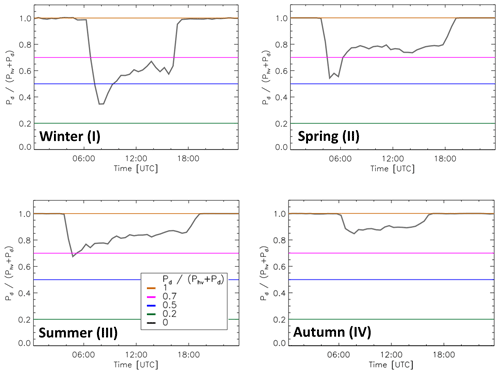Figure 9The median ratio of dark to total atmospheric OH production rates during four JULIAC periods (grey lines). Pd is the calculated reaction rate of HO2 with NO (Reaction R4), and Phv is the OH production rate from the photolysis of ozone (Reactions R1 and R2) and HONO (Reaction R3). For the calculations, measured quantities (300 s averaged) were used (Table 2). The coloured horizontal lines denote the same ratios of dark to total OH production rates as used in Figs. 7 and 8.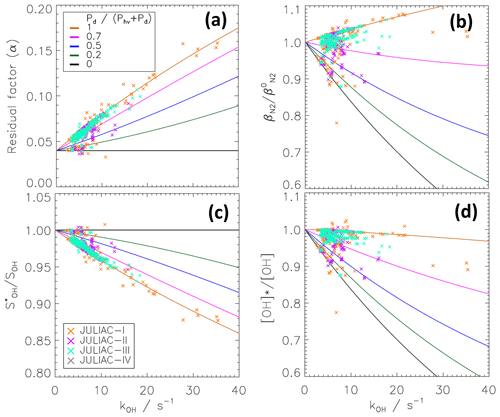Figure 10Modelled kOH dependence of the CMR properties for the atmospheric chemical conditions during the JULIAC periods in different seasons of 2019. Here, the model for a homogeneously mixed scavenger was applied. See Fig. 7 for further explanations of (a–c). Panel (d) shows the ratio of corrected to uncorrected ambient OH concentrations, if the kOH dependence of α and ${\mathit{\beta }}_{{\mathrm{N}}_{\mathrm{2}}}$ is taken into account.

Although during the winter season (I) the influence on the calculated ambient OH signal could reach 12%, on average, the combined influence on OH concentration is 2 % for the JULIAC data set. If the corresponding corrections were applied, the amount of useful OH radical data would be reduced by 20 % because not all data (e.g. HO2, NO and HONO) needed for corrections were always available. For this reason, no additional corrections were applied to the OH radical JULIAC data set.

### 4.2.2 OH measurement comparison

The combined OH data set measured during the JULIAC campaign by DOAS and LIF-CMR was used for an OH measurement comparison in ambient air. Only summertime (August) data are used because OH concentrations for the other JULIAC periods were either close to the limit of detection of the instruments (winter and autumn) or the DOAS instrument was not operated (spring). In general, the measurements by LIF and DOAS agree well within their combined 1σ accuracies of 18 % and 6.5 %, respectively (Fig. 11). An exception is the heat wave period between 22 and 26 August, when the LIF measurements were systematically higher than the DOAS measurements by about 25 %. The reason for the discrepancy is not clear, as no issue with either instrument was found. A linear regression analysis, which considers the precision of both instruments, is done using the fitexy algorithm (Press, 1992). The scatterplot of the OH data and the regression line forced through the origin are shown in Fig. 12. The slope of the regression line is 1.11±0.02, where the deviation from unity can be explained by the instrumental accuracies, and therefore no significant systematic error from the calibration with the CMR was found. To see the statistical error, the residuals of the OH concentrations by LIF obtained from the difference between the measured OH concentration and the linear fit are shown in Fig. S2. The residuals scatter symmetrically around zero without curvature, which implies that the linear fit is suitable to describe the relationship between the two OH measurements. Similar good agreement was found in previous OH intercomparisons between the DOAS and FZJ-LIF instruments, when the LIF instrument used wavelength modulation only and data were corrected for the known ozone interference. These intercomparisons were performed in photochemical degradation experiments in SAPHIR for a wide range of VOCs and chemical conditions (Schlosser et al., 2007, 2009; Fuchs et al., 2012a; Novelli et al., 2018; Rolletter et al., 2019; Novelli et al., 2020) and in a field campaign in relatively clean air in northeastern Germany (Hofzumahaus et al., 1998).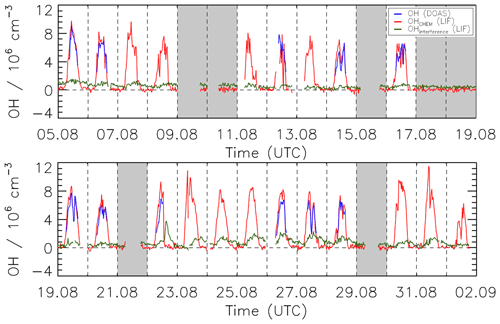Figure 11Time series of OH concentrations measured by LIF-CMR (red line) and DOAS (blue line) during the summer period of the JULIAC campaign. The green line shows the equivalent OH concentration of the interference signal in the LIF system. All data points are 30 min averages. Vertical dashed lines denote midnight. Grey-shaded areas indicate when the chamber roof was closed. Data gaps occurred when measurements were stopped for calibrating instruments.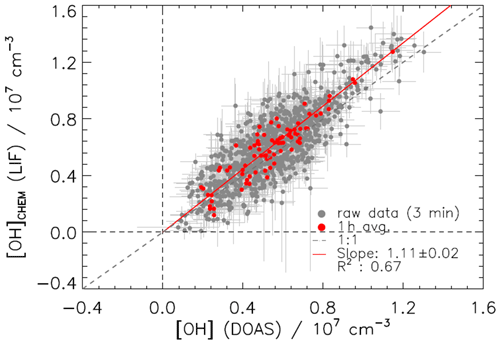Figure 12Correlation plot between OH concentrations measured by the FZJ-LIF-CMR and the DOAS instruments during the summer period of the JULIAC campaign except for the heat wave period between 22 and 26 August. The 1436 data points are averaged over 3 min and 1 h. The red line is a linear fit of the 3 min data set weighted with the statistical errors of both instruments and forced to the origin. Vertical bars denote the 1σ precision of the measured data points.

### 4.2.3 OH interferences

The time series of the OH interference measured by chemical modulation during the summer (III) season is presented in Fig. 11. The interference shows diurnal variations with minimum values at night and maximum values around midday. The median diurnal variation of the interference for the days from 5 August to 2 September has a maximum daytime value of 0.9×106 cm−3 and a minimum nighttime value of 0.4×106 cm−3. The highest value of 2×106 cm−3 occurred in a heat wave from 22 to 29 August, when the daytime air temperature and ozone reached 40 C and 100 ppbv, respectively. At the same time, the total OH reactivity reached the highest values (19 s−1) with a contribution of reactivity from organic compounds kVOC of up to 14 s−1. During the other seasons, interfering signals at daytime were lower with maximum values of 1×106 cm−3 (Figs. S3–S5).

Figure 13 compares the OH interferences measured during all four periods of JULIAC with the expected interference from the laser photolysis of ozone. The expected ozone interference is calculated by Eq. (19) using the average result from the three experiments in Fig. 5. Please note that there is a slight curvature in the parametrization (Fig. 13) due to the water-quenching effect of the OH radical in the detection cell (Holland et al., 2003) by the large range of the water vapour mixing ratio during the JULIAC campaign. Over the whole range of atmospheric conditions, the average measured interferences can be explained by the known ozone–water interference within the uncertainty of the parametrization. Over the whole range of atmospheric conditions, the average measured interferences can be explained by the known ozone–water interference within the uncertainty of the parametrization. The maximum difference between measured and known interferences is $\left(\mathrm{3.4}±\mathrm{2.5}\right)×{\mathrm{10}}^{\mathrm{5}}$ cm−3 for the highest ozone and water vapour concentrations. This difference is well below the limit of detection of the LIF-CMR technique (7×105 cm−3). Thus, there is no evidence for a significant, unexplained OH interference for the atmospheric conditions during the JULIAC campaign (Table 3). Note that the contribution of NO3 to the interfering signal was negligible for the entire duration of the campaign due to the low NO3 concentrations that were maximum 10 pptv. Although it is possible that the chemical conditions experienced during the JULIAC campaign were not ideal to observe large interferences as reported in other studies (Mao et al., 2012; Hens et al., 2014; Novelli et al., 2014a; Feiner et al., 2016; Lew et al., 2020), the good agreement between the CMR-LIF-FZJ and the DOAS instruments indicates that the CMR is correctly implemented. It provides interference-free OH radical concentrations by separating the atmospheric OH signal from the OH radical produced within the detection cell.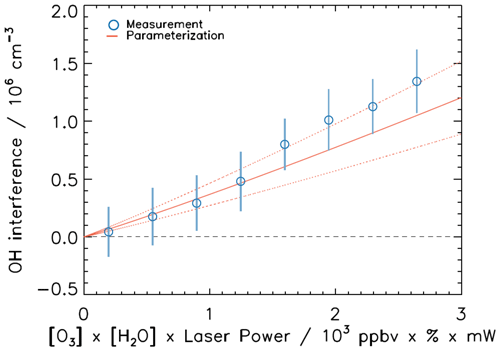Figure 13Averaged OH interference measured during all JULIAC periods (vertical bars are standard deviations of the bin averaged data) compared to a parameterization (Eq. 19 and Sect. 3.5.1) determined in laboratory experiments. The red dotted lines represent the uncertainty of the parameterization. Each bin is averaged by more than 100 data points.

5 Discussion

The experimental tests in synthetic (Sect. 3) and in ambient air during the JULIAC campaign (Sect. 4) demonstrate that the new chemical modulation system of the FZJ-LIF instrument is suitable for measurements of interference-free ambient OH concentrations and internal OH interferences. The theoretically expected dependence of the OH scavenging efficiency and OH transmission on ambient conditions could not be tested. The effects during the conditions of the JULIAC campaign were too small to be clearly detected within the precision of the measurements. Thus, for atmospheric OH reactivities below 15 s−1, it was sufficient to use the CMR parameters that were characterized in synthetic air.

In other field campaigns with higher OH reactivities (>30 s−1), for example in strongly polluted urban air or in forest environments, the accurate evaluation of OH measurement with the CMR could be more challenging. As shown in Figs. 7a and 8a, the residual factor α could significantly increase at high OH reactivities. If not properly corrected, this leads to an overestimation of the calculated interference (Fig. 7d). A systematic underestimation of ambient OH signals on the order of (15–25) % would be possible (Fig. 7c). The sensitivity to kOH can be alleviated by applying higher scavenger reactivities. Some other research groups used much higher scavenger concentrations for their chemical modulation systems (Table 1) with reactivities that are a factor of 5 to 100 higher than in our work (Mao et al., 2012; Novelli et al., 2014a; Rickly and Stevens, 2018; Woodward-Massey et al., 2020). For scavenger reactivities above 10 000 s−1, we expect that the influence of atmospheric kOH on the scavenging efficiency becomes completely negligible. However, different from other groups who did not observe internal OH scavenging in their instruments, we find indications of internal scavenging by 5 % when the injected propane concentration is increased by only a factor of 3.8 (Fig. 3d). To avoid internal scavenging, which would underestimate the instrumental interference, we kept the scavenger concentration low. Only one other LIF group reported detectable internal scavenging of approximately 5–10 % but for a scavenger reactivity of 30 000 s−1 (Woodward-Massey et al., 2020). Currently, it is not clear why the FZJ instrument seems to be more sensitive to internal scavenging than other instruments.

A more serious challenge is the variability of the OH transmission with ambient conditions when no scavenger is added. The transmission (${\mathit{\beta }}_{{\mathrm{N}}_{\mathrm{2}}}$) needs to be known in order to calculate the ambient OH concentration from the retrieved interference-free ambient OH signal (Eq. 6). The transmission in the mode without any scavenger (N2 mode) depends on the wall loss rate, the atmospheric OH reactivity and the transit time through the chemical modulation reactor. The possible influence of atmospheric kOH on the transmission of the FZJ instrument can be seen in Fig. 7b. While the impact for the JULIAC conditions is relatively small (Sect. 4.2.1), a considerable impact would be expected, for example, in a pristine environment like in the Amazonian rain forest. Here, OH reactivities up to 70 s−1 were reported (Sinha et al., 2008), while the dark OH production from the reaction of HO2 with NO can be small compared to the total OH production rate. Novelli et al. (2020) estimated the chemical regeneration of OH by Reaction (R4) to be 10 % in an isoprene emitting forest at 10 pptv NO, which according to the steady-state condition (Eq. 20) would correspond to a ${P}_{\mathrm{d}}/\left({P}_{hv}+{P}_{\mathrm{d}}\right)$ ratio of 0.1. For such conditions, the kinetic model for the CMR predicts a decrease of the OH transmission by a factor of 2.5–3 at kOH=70 s−1. A large correction is required to account for this effect. Novelli et al. (2020) also point out that additional OH regeneration occurs by isomerization of isoprene peroxy radicals, which increases the non-photolytic regeneration rate to about 50 % of the OH loss rate. With a ${P}_{\mathrm{d}}/\left({P}_{hv}+{P}_{\mathrm{d}}\right)$ ratio of 0.5, the predicted decrease of the CMR transmission is still a factor of 1.5. For this particular environment, the chemical mechanism for OH regeneration including processes like RO2 isomerization needs to be known to calculate the CMR transmission.

One possibility to reduce the sensitivity of the transmission with respect to kOH is to shorten the transit time in the CMR. The instrument by the MPIC group (Novelli et al., 2014a) uses a residence time which is a factor of 3–4 smaller than in our system. A similar time reduction in the FZJ system would lead to much smaller variations of ${\mathit{\beta }}_{{\mathrm{N}}_{\mathrm{2}}}$ (<10 %) at high kOH values. The main disadvantage is the requirement to draw a large airflow in the order of 100 slpm through the CMR. Such a high flow rate has several problems. The current radical source provides a maximum of 30 slpm of calibration gas and would need to be newly designed. Second, delivering high flows of clean (synthetic) air for calibration and characterization is expensive. Third, the application in SAPHIR would not be possible because this high flow rate exceeds the total amount of air that can be taken from the chamber during typical operation.

Another solution to quantify the impact of ambient conditions on the OH transmission is to measure the transmission in situ in the field by taking measurements of OH with and without the CMR in the same air. This directly provides the transmission for the prevailing conditions but works only reasonably, if OH measurements are interference free. With interference from internal OH, the transmission is overestimated. In order to track changes of the CMR transmission with ambient kOH and diurnal variation of ${P}_{\mathrm{d}}/{P}_{hv}$, the transmission needs to be frequently determined. This complicates the automatic measuring operation. A number of groups determined the CMR transmission in the field by this approach but did not discuss the possible influence of ambient conditions on their results (Table 1). Mao et al. (2012) reported negligible OH loss in field tests, and Woodward-Massey et al. (2020) found less than a 5 % sensitivity reduction in ambient air. Both systems used transit times similar to our system. Contrarily, the MPIC instrument reported lower transmissions of 73 % during daytime for a much shorter residence time. Because of the sparse information about the characteristics of chemical modulation reactors in literature, it is not possible to perform more quantitative comparisons.

Different CMR prototypes built in Jülich were tested with the Peking University LIF instrument (PKU-LIF) in three field campaigns in China (Tan et al., 2017, 2018, 2019). The reactor diameter and total airflow were the same as in this work, but different reactor lengths, injector types, scavenger concentrations and injection flow rates were applied (Table 1). The tests were performed under different ambient conditions in summer 2014 in Wangdu, south of Beijing (Tan et al., 2017); in autumn 2014 in Heshan in the Pearl River Delta in southern China (Tan et al., 2019); and in winter 2016 in Huairou, north of Beijing (Tan et al., 2018). In each case, the CMR was occasionally mounted for a few hours on the LIF instrument to investigate possible OH interferences. The scavenging efficiency was determined in the field using an OH calibration source. Similar to what is observed during JULIAC, no significant unknown OH interference signals were detected during the field experiments with the exception of the campaign in Wangdu (Tan et al., 2017), where a relatively high level of isoprene (up to 3 ppbv) was observed. Here, unexplained OH signals equivalent to $\left(\mathrm{0.5}-\mathrm{1}\right)×{\mathrm{10}}^{\mathrm{6}}$ cm−3 were found with an experimental 1σ uncertainty of 0.5×106 cm−3. Around noontime, these signals were less than 10 % of the ambient OH signals. However, the magnitude of the interference was small compared to the field observations with other LIF instruments in forested environments (Mao et al., 2012; Hens et al., 2014; Novelli et al., 2014a; Feiner et al., 2016; Lew et al., 2020). The evaluation did not take into account the possible dependence on ambient conditions that is discussed in Sect. 4. At daytime with OH reactivities of about 15 s−1, about half of the unknown interference could have been an artefact due to the unaccounted influence of the atmospheric OH reactivity on the scavenging efficiency, but at nighttime a correction for this influence does not make a difference. Tan et al. (2017) reported also that the sensitivity loss of the OH detection with the CMR of only 5 %. This result was derived from measurements with and without the CMR using an OH calibration source with a longer flow tube (Fuchs et al., 2012a) that does not provide a uniform OH concentration due to its flow profile. CMR transmission measurements with this radical source and with the plug flow radical source used in this work (Sect. 3.1) show that the transmission reported by Tan et al. (2017) needs to be corrected from 0.95 to 0.7. The corrected value is in better agreement with the result for the CMR in the present work. The expected small changes do not have any impact on the main findings of the study, which basically used [OH]WAVE corrected for the known ozone interference.

6 Summary and conclusions

A chemical modulation reactor (CMR) for the measurement of interference-free OH radicals with a LIF system was characterized and implemented in the FZJ-LIF instrument. Experiments in the SAPHIR chamber allowed for a comprehensive investigation of possible interferences for a wide range of chemical and meteorological conditions.

Several laboratory characterization tests with an OH calibration source enabled an optimization of the operational parameters of the CMR. The reactor has an OH transmission of 64 % at a sample flow of 21 slpm. It was found that a concentration of 19 ppmv of propane by injection with 500 sccm of carrier flow provides efficient mixing and enough OH radical scavenging (96 %) without any significant removal of radicals in the low-pressure detection cell (3 %). The total residence time in the reactor is 18 ms, and the reaction time for the scavenger is 11 ms.

A comparison with the DOAS instrument in the SAPHIR chamber with humidified synthetic air provided very good agreement to better than 10 % between the two measurements. Interferences from the laser photolysis of ozone in humid air and from NO3 reactions in the detection system known from previous studies with the FZJ-LIF were revisited for the instrument with the CMR in the SAPHIR chamber. The photolytic ozone–water interference is equivalent to an OH concentration of 3.4×105 cm−3 for 50 ppbv O3 and a 1 % water vapour mixing ratio, in good agreement with what has previously been found (Holland et al., 2003; Schlosser et al., 2009; Fuchs et al., 2012a). An interference in the presence of NO3 was equivalent to an OH concentration of 0.6×105 cm−3 per 10 pptv NO3, a factor of 2 smaller than what has previously been measured (Fuchs et al., 2016). However, this is not atmospherically relevant.

Four intensive measurement campaigns were performed in four different seasons with sampling ambient air into the SAPHIR chamber (JULIAC). The interferences measured in the summer season had the median diurnal variation of the interference with a maximum value of 0.9×106 cm−3 during daytime and a minimum value of 0.4×106 cm−3 at night. The highest interference equivalent to an OH concentration of 2×106 cm−3 occurred in a heat wave from 22 to 29 August, when the air temperature and ozone increased to 40 C and 100 ppbv, respectively. This interference could be fully explained by the known ozone interference. No additional unknown interference was observed. Comparison of OH measurements between LIF-CMR and DOAS in ambient air during the JULIAC summer period showed good agreement within measurement uncertainties, with LIF being 13 % larger than DOAS.

A simple kinetic model is presented in this work to estimate the possible influence of ambient chemical conditions on the OH transmission and scavenging efficiency in the CMR. When ambient air is sampled, OH reactions with air pollutants at high OH reactivities begin to compete with OH loss by wall reactions and scavenging. This perturbation depends also on the non-photolytic atmospheric OH production, most importantly the reaction of HO2 with NO, which continues when the sampled ambient air enters the chemical modulation reactor. A correction requires a suite of trace gas measurements (HONO, NO, HO2, kOH and photolysis frequencies). It is found that, for the chemical conditions investigated in this study, the impact on the evaluated OH concentration was small (2 %). In other environments, in particular those with high VOC reactivities and low NOx, OH loss reactions with atmospheric constituents can produce serious perturbations on the transmission and the scavenging efficiency of the CMR unless very high flow rates are used like in the instrument of MPIC. For residence times on the order of 20 ms, as used here and in some instruments of other groups, ambient OH can be depleted in the CMR by more than a factor of 2 by atmospheric reactants. Such perturbations need to be investigated for the specific chemical conditions to evaluate if corrections need to be applied. This means that chemical modulation, which was developed to eliminate interferences in ambient OH measurements, itself can be subject to interferences that depend on ambient atmospheric conditions. In addition, the OH detection sensitivity and precision as well as time resolution are worse compared to the system without the CMR. So far, for experiments in the SAPHIR chamber, OH radical measurement by the FZJ-LIF has shown good agreement with measurements by DOAS without the need of chemical modulation (Schlosser et al., 2007, 2009; Fuchs et al., 2012a; Novelli et al., 2018; Rolletter et al., 2019; Novelli et al., 2020). However, it is not possible to exclude the presence of an unknown interference for all the possible environments. The continued application of chemical modulation will therefore remain an important tool for the detection of OH by LIF.

Appendix A

Table A1Specification of CMR parameters used in this study.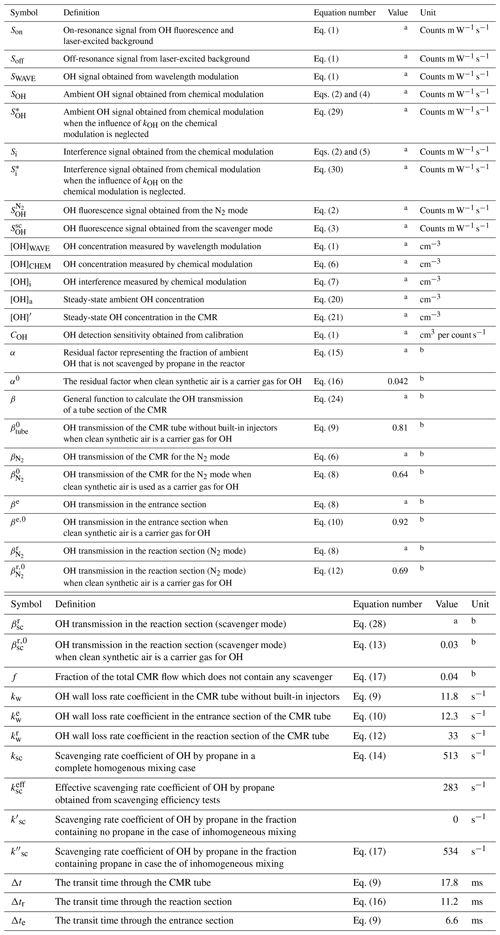a Variable. b Dimensionless.

Data availability

The data from the JULIAC campaign used in this study are available on the Forschungszentrum Jülich data storage page (https://doi.org/10.26165/JUELICH-DATA/GK7PJQ, Cho et al., 2021).

Supplement

Author contributions

AH designed the JULIAC campaign and organized it together with HF and FH. CC performed the laboratory experiments, analysed the data, and wrote the paper together with AN and AH. All co-authors contributed with data and to the discussion of the paper.

Competing interests

The authors declare that they have no conflict of interest.

Acknowledgements

We thank Birger Bohn for the provision of photolysis frequencies for the JULIAC campaign and Zhaofeng Tan for the discussion about the CMR conditions during the field campaigns in China.

Financial support

This research has been supported by the European Research Council of the European Commission under the European Union’s Horizon 2020 research and innovation programme (SARLEP (grant no. 681529)).

The article processing charges for this open-access
publication were covered by a Research
Centre of the Helmholtz Association.

Review statement

This paper was edited by Glenn Wolfe and reviewed by two anonymous referees.

References

Atkinson, R., Baulch, D. L., Cox, R. A., Crowley, J. N., Hampson, R. F., Hynes, R. G., Jenkin, M. E., Rossi, M. J., and Troe, J.: Evaluated kinetic and photochemical data for atmospheric chemistry: Volume I - gas phase reactions of Ox, HOx, NOx and SOx species, Atmos. Chem. Phys., 4, 1461–1738, https://doi.org/10.5194/acp-4-1461-2004, 2004.

Atkinson, R., Baulch, D. L., Cox, R. A., Crowley, J. N., Hampson, R. F., Hynes, R. G., Jenkin, M. E., Rossi, M. J., Troe, J., and IUPAC Subcommittee: Evaluated kinetic and photochemical data for atmospheric chemistry: Volume II – gas phase reactions of organic species, Atmos. Chem. Phys., 6, 3625–4055, https://doi.org/10.5194/acp-6-3625-2006, 2006.

Berndt, T.: Formation of carbonyls and hydroperoxyenals (HPALDs) from the OH radical reaction of isoprene for low-NOx conditions: influence of temperature and water vapour content, J. Atmos. Chem., 69, 253–272, https://doi.org/10.1007/s10874-012-9245-2, 2012.

Berndt, T., Hyttinen, N., Herrmann, H., and Hansel, A.: First oxidation products from the reaction of hydroxyl radicals with isoprene for pristine environmental conditions, Comm. Chem., 2, 21, https://doi.org/10.1038/s42004-019-0120-9, 2019.

Berresheim, H., Elste, T., Plass-Dülmer, C., Eiseleb, F. L., and Tannerb, D. J.: Chemical ionization mass spectrometer for long-term measurements of atmospheric OH and H2SO4, Int. J. Mass Spectrom., 202, 91–109, https://doi.org/10.1016/S1387-3806(00)00233-5, 2000.

Bohn, B. and Zilken, H.: Model-aided radiometric determination of photolysis frequencies in a sunlit atmosphere simulation chamber, Atmos. Chem. Phys., 5, 191–206, https://doi.org/10.5194/acp-5-191-2005, 2005.

Bohn, B., Rohrer, F., Brauers, T., and Wahner, A.: Actinometric measurements of NO2 photolysis frequencies in the atmosphere simulation chamber SAPHIR, Atmos. Chem. Phys., 5, 493–503, https://doi.org/10.5194/acp-5-493-2005, 2005.

Brune, W. H., Baier, B. C., Thomas, J., Ren, X., Cohen, R. C., Pusede, S. E., Browne, E. C., Goldstein, A. H., Gentner, D. R., Keutsch, F. N., Thornton, J. A., Harrold, S., Lopez-Hilfiker, F. D., and Wennberg, P. O.: Ozone production chemistry in the presence of urban plumes, Faraday Discuss., 189, 169–189, https://doi.org/10.1039/C5FD00204D, 2016.

Carslaw, K. S., Boucher, O., Spracklen, D. V., Mann, G. W., Rae, J. G. L., Woodward, S., and Kulmala, M.: A review of natural aerosol interactions and feedbacks within the Earth system, Atmos. Chem. Phys., 10, 1701–1737, https://doi.org/10.5194/acp-10-1701-2010, 2010.

Cho, C., Hofzumahaus, A., Fuchs, H., Dorn, H.-P., Glowania, M., Holland, F., Rohrer, F., Vardhan, V., Kiendler-Scharr, A., Wahner, A., and Novelli, A.: Characterization and application of a chemical modulation reactor during the JULIAC campaign, V1, Jülich DATA, https://doi.org/10.26165/JUELICH-DATA/GK7PJQ, 2021.

Crounse, J. D., Paulot, F., Kjaergaard, H. G., and Wennberg, P. O.: Peroxy radical isomerization in the oxidation of isoprene, Phys. Chem. Chem. Phys., 13, 13607–13613, https://doi.org/10.1039/C1CP21330J, 2011.

Crounse, J. D., Knap, H. C., Ørnsø, K. B., Jørgensen, S., Paulot, F., Kjaergaard, H. G., and Wennberg, P. O.: Atmospheric Fate of Methacrolein. 1. Peroxy Radical Isomerization Following Addition of OH and O2, J. Phys. Chem. A, 116, 5756–5762, https://doi.org/10.1021/jp211560u, 2012.

da Silva, G., Graham, C., and Wang, Z.-F.: Unimolecular β-hydroxyperoxy radical decomposition with OH recycling in the photochemical oxidation of isoprene, Environ. Sci. Technol., 44, 250–256, https://doi.org/10.1021/es900924d, 2010.

Dorn, H. P., Neuroth, R., and Hofzumahaus, A.: Investigation of OH absorption cross sections of rotational transitions in the A26+, ν0=0<X25, ν00=0 band under atmospheric conditions: Implications for tropospheric long-path absorption measurements, J. Geophys. Res., 100, 7397–7409, 1995.

Dorn, H.-P., Apodaca, R. L., Ball, S. M., Brauers, T., Brown, S. S., Crowley, J. N., Dubé, W. P., Fuchs, H., Häseler, R., Heitmann, U., Jones, R. L., Kiendler-Scharr, A., Labazan, I., Langridge, J. M., Meinen, J., Mentel, T. F., Platt, U., Pöhler, D., Rohrer, F., Ruth, A. A., Schlosser, E., Schuster, G., Shillings, A. J. L., Simpson, W. R., Thieser, J., Tillmann, R., Varma, R., Venables, D. S., and Wahner, A.: Intercomparison of NO3 radical detection instruments in the atmosphere simulation chamber SAPHIR, Atmos. Meas. Tech., 6, 1111–1140, https://doi.org/10.5194/amt-6-1111-2013, 2013.

Dubey, M. K., Hanisco, T. F., Wennberg, P. O., and Anderson, J. G.: Monitoring potential photochemical interference in laser-induced fluorescence measurements of atmospheric OH, Geophys. Res. Lett., 23, 3215–3218, https://doi.org/10.1029/96gl03008, 1996.

Eisele, F. L., and Tanner, D. J.: Ion-assisted tropospheric OH measurements, J. Geophys. Res.-Atmos., 96, 9295–9308, https://doi.org/10.1029/91JD00198, 1991.

Feiner, P. A., Brune, W. H., Miller, D. O., Zhang, L., Cohen, R. C., Romer, P. S., Goldstein, A. H., Keutsch, F. N., Skog, K. M., Wennberg, P. O., Nguyen, T. B., Teng, A. P., DeGouw, J., Koss, A., Wild, R. J., Brown, S. S., Guenther, A., Edgerton, E., Baumann, K., and Fry, J. L.: Testing atmospheric oxidation in an Alabama forest, J. Atmos. Sci., 73, 4699–4710, https://doi.org/10.1175/jas-d-16-0044.1, 2016.

Fuchs, H., Holland, F., and Hofzumahaus, A.: Measurement of tropospheric RO2 and HO2 radicals by a laser-induced fluorescence instrument, Rev. Sci. Instrum., 79, 084104, https://doi.org/10.1063/1.2968712, 2008.

Fuchs, H., Dubé, W. P., Lerner, B. M., Wagner, N. L., Williams, E. J., and Brown, S. S.: A Sensitive and Versatile Detector for Atmospheric NO2 and NOx Based on Blue Diode Laser Cavity Ring-Down Spectroscopy, Environ. Sci. Technol., 43, 7831–7836, https://doi.org/10.1021/es902067h, 2009.

Fuchs, H., Ball, S. M., Bohn, B., Brauers, T., Cohen, R. C., Dorn, H.-P., Dubé, W. P., Fry, J. L., Häseler, R., Heitmann, U., Jones, R. L., Kleffmann, J., Mentel, T. F., Müsgen, P., Rohrer, F., Rollins, A. W., Ruth, A. A., Kiendler-Scharr, A., Schlosser, E., Shillings, A. J. L., Tillmann, R., Varma, R. M., Venables, D. S., Villena Tapia, G., Wahner, A., Wegener, R., Wooldridge, P. J., and Brown, S. S.: Intercomparison of measurements of NO2 concentrations in the atmosphere simulation chamber SAPHIR during the NO3Comp campaign, Atmos. Meas. Tech., 3, 21–37, https://doi.org/10.5194/amt-3-21-2010, 2010a.

Fuchs, H., Brauers, T., Dorn, H.-P., Harder, H., Häseler, R., Hofzumahaus, A., Holland, F., Kanaya, Y., Kajii, Y., Kubistin, D., Lou, S., Martinez, M., Miyamoto, K., Nishida, S., Rudolf, M., Schlosser, E., Wahner, A., Yoshino, A., and Schurath, U.: Technical Note: Formal blind intercomparison of HO2 measurements in the atmosphere simulation chamber SAPHIR during the HOxComp campaign, Atmos. Chem. Phys., 10, 12233–12250, https://doi.org/10.5194/acp-10-12233-2010, 2010b.

Fuchs, H., Bohn, B., Hofzumahaus, A., Holland, F., Lu, K. D., Nehr, S., Rohrer, F., and Wahner, A.: Detection of HO2 by laser-induced fluorescence: calibration and interferences from RO2 radicals, Atmos. Meas. Tech., 4, 1209–1225, https://doi.org/10.5194/amt-4-1209-2011, 2011.

Fuchs, H., Dorn, H.-P., Bachner, M., Bohn, B., Brauers, T., Gomm, S., Hofzumahaus, A., Holland, F., Nehr, S., Rohrer, F., Tillmann, R., and Wahner, A.: Comparison of OH concentration measurements by DOAS and LIF during SAPHIR chamber experiments at high OH reactivity and low NO concentration, Atmos. Meas. Tech., 5, 1611–1626, https://doi.org/10.5194/amt-5-1611-2012, 2012a.

Fuchs, H., Simpson, W. R., Apodaca, R. L., Brauers, T., Cohen, R. C., Crowley, J. N., Dorn, H.-P., Dubé, W. P., Fry, J. L., Häseler, R., Kajii, Y., Kiendler-Scharr, A., Labazan, I., Matsumoto, J., Mentel, T. F., Nakashima, Y., Rohrer, F., Rollins, A. W., Schuster, G., Tillmann, R., Wahner, A., Wooldridge, P. J., and Brown, S. S.: Comparison of N2O5 mixing ratios during NO3Comp 2007 in SAPHIR, Atmos. Meas. Tech., 5, 2763–2777, https://doi.org/10.5194/amt-5-2763-2012, 2012b.

Fuchs, H., Hofzumahaus, A., Rohrer, F., Bohn, B., Brauers, T., Dorn, H. P., Häseler, R., Holland, F., Kaminski, M., Li, X., Lu, K., Nehr, S., Tillmann, R., Wegener, R., and Wahner, A.: Experimental evidence for efficient hydroxyl radical regeneration in isoprene oxidation, Nat. Geosci., 6, 1023–1026, https://doi.org/10.1038/ngeo1964, 2013.

Fuchs, H., Acir, I.-H., Bohn, B., Brauers, T., Dorn, H.-P., Häseler, R., Hofzumahaus, A., Holland, F., Kaminski, M., Li, X., Lu, K., Lutz, A., Nehr, S., Rohrer, F., Tillmann, R., Wegener, R., and Wahner, A.: OH regeneration from methacrolein oxidation investigated in the atmosphere simulation chamber SAPHIR, Atmos. Chem. Phys., 14, 7895–7908, https://doi.org/10.5194/acp-14-7895-2014, 2014.

Fuchs, H., Tan, Z., Hofzumahaus, A., Broch, S., Dorn, H.-P., Holland, F., Künstler, C., Gomm, S., Rohrer, F., Schrade, S., Tillmann, R., and Wahner, A.: Investigation of potential interferences in the detection of atmospheric ROx radicals by laser-induced fluorescence under dark conditions, Atmos. Meas. Tech., 9, 1431–1447, https://doi.org/10.5194/amt-9-1431-2016, 2016.

Fuchs, H., Novelli, A., Rolletter, M., Hofzumahaus, A., Pfannerstill, E. Y., Kessel, S., Edtbauer, A., Williams, J., Michoud, V., Dusanter, S., Locoge, N., Zannoni, N., Gros, V., Truong, F., Sarda-Esteve, R., Cryer, D. R., Brumby, C. A., Whalley, L. K., Stone, D., Seakins, P. W., Heard, D. E., Schoemaecker, C., Blocquet, M., Coudert, S., Batut, S., Fittschen, C., Thames, A. B., Brune, W. H., Ernest, C., Harder, H., Muller, J. B. A., Elste, T., Kubistin, D., Andres, S., Bohn, B., Hohaus, T., Holland, F., Li, X., Rohrer, F., Kiendler-Scharr, A., Tillmann, R., Wegener, R., Yu, Z., Zou, Q., and Wahner, A.: Comparison of OH reactivity measurements in the atmospheric simulation chamber SAPHIR, Atmos. Meas. Tech., 10, 4023–4053, https://doi.org/10.5194/amt-10-4023-2017, 2017a.

Fuchs, H., Tan, Z., Lu, K., Bohn, B., Broch, S., Brown, S. S., Dong, H., Gomm, S., Häseler, R., He, L., Hofzumahaus, A., Holland, F., Li, X., Liu, Y., Lu, S., Min, K.-E., Rohrer, F., Shao, M., Wang, B., Wang, M., Wu, Y., Zeng, L., Zhang, Y., Wahner, A., and Zhang, Y.: OH reactivity at a rural site (Wangdu) in the North China Plain: contributions from OH reactants and experimental OH budget, Atmos. Chem. Phys., 17, 645–661, https://doi.org/10.5194/acp-17-645-2017, 2017b.

Fuchs, H., Albrecht, S., Acir, I., Bohn, B., Breitenlechner, M., Dorn, H.-P., Gkatzelis, G. I., Hofzumahaus, A., Holland, F., Kaminski, M., Keutsch, F. N., Novelli, A., Reimer, D., Rohrer, F., Tillmann, R., Vereecken, L., Wegener, R., Zaytsev, A., Kiendler-Scharr, A., and Wahner, A.: Investigation of the oxidation of methyl vinyl ketone (MVK) by OH radicals in the atmospheric simulation chamber SAPHIR, Atmos. Chem. Phys., 18, 8001–8016, https://doi.org/10.5194/acp-18-8001-2018, 2018.

Griffith, S. M., Hansen, R. F., Dusanter, S., Michoud, V., Gilman, J. B., Kuster, W. C., Veres, P. R., Graus, M., de Gouw, J. A., Roberts, J., Young, C., Washenfelder, R., Brown, S. S., Thalman, R., Waxman, E., Volkamer, R., Tsai, C., Stutz, J., Flynn, J. H., Grossberg, N., Lefer, B., Alvarez, S. L., Rappenglueck, B., Mielke, L. H., Osthoff, H. D., and Stevens, P. S.: Measurements of hydroxyl and hydroperoxy radicals during CalNex-LA: Model comparisons and radical budgets, J. Geophys. Res.-Atmos., 121, 4211–4232, https://doi.org/10.1002/2015jd024358, 2016.

Hausmann, M., Brandenburger, U., Brauers, T., and Dorn, H.-P.: Detection of tropospheric OH radicals by long-path differential optical-absorption spectroscopy: Experimental setup, accuracy, and precision, J. Geophys. Res., 102, 16011–16022, 1997.

Hens, K., Novelli, A., Martinez, M., Auld, J., Axinte, R., Bohn, B., Fischer, H., Keronen, P., Kubistin, D., Nölscher, A. C., Oswald, R., Paasonen, P., Petäjä, T., Regelin, E., Sander, R., Sinha, V., Sipilä, M., Taraborrelli, D., Tatum Ernest, C., Williams, J., Lelieveld, J., and Harder, H.: Observation and modelling of HOx radicals in a boreal forest, Atmos. Chem. Phys., 14, 8723–8747, https://doi.org/10.5194/acp-14-8723-2014, 2014.

Hofzumahaus, A., Aschmutat, U., Heßling, M., Holland, F., and Ehhalt, D. H.: The measurement of tropospheric OH radicals by laser-induced fluorescence spectroscopy during the POPCORN Field Campaign, Geophys. Res. Lett., 23, 2541–2544, https://doi.org/10.1029/96gl02205, 1996.

Hofzumahaus, A., Aschmutat, U., Brandenburger, U., Brauers, T., Dorn, H. P., Hausmann, M., Heßling, M., Holland, F., Plass-Dülmer, C., and Ehhalt, D. H.: Intercomparison of Tropospheric OH Measurements by Different Laser Techniques during the POPCORN Campaign 1994, J. Atmos. Chem., 31, 227–246, https://doi.org/10.1023/A:1006014707617, 1998.

Hofzumahaus, A., Rohrer, F., Lu, K., Bohn, B., Brauers, T., Chang, C.-C., Fuchs, H., Holland, F., Kita, K., Kondo, Y., Li, X., Lou, S., Shao, M., Zeng, L., Wahner, A., and Zhang, Y.: Amplified trace gas removal in the troposphere, Science, 324, 1702–1704, https://doi.org/10.1126/science.1164566, 2009.

Hofzumahaus, A. and Heard, D. H.: Assessment of local HOx and ROx measurement techniques: achivements, challenges, and future directions - Outcomes of the 2015 international HOx workshop, Forschungszentrum Jülich, Jülich, 23–25 March 2015, 20–21, 2016.

Holland, F., Aschmutat, U., Heßling, M., Hofzumahaus, A., and Ehhalt, D. H.: Highly Time Resolved Measurements of OH during POPCORN Using Laser-Induced Fluorescence Spectroscopy, in: Atmospheric Measurements during POPCORN – Characterisation of the Photochemistry over a Rural Area, edited by: Rudolph, J. and Koppmann, R., Springer Netherlands, Dordrecht, 205–225, 1998.

Holland, F., Hofzumahaus, A., Schäfer, J., Kraus, A., and Pätz, H.-W.: Measurements of OH and HO2 radical concentrations and photolysis frequencies during BERLIOZ, J. Geophys. Res.-Atmos., 108, 8246, https://doi.org/10.1029/2001jd001393, 2003.

Kleffmann, J., Lörzer, J. C., Wiesen, P., Kern, C., Trick, S., Volkamer, R., Rodenas, M., and Wirtz, K.: Intercomparison of the DOAS and LOPAP techniques for the detection of nitrous acid (HONO), Atmos. Environ., 40, 3640–3652, https://doi.org/10.1016/j.atmosenv.2006.03.027, 2006.

Kubistin, D., Harder, H., Martinez, M., Rudolf, M., Sander, R., Bozem, H., Eerdekens, G., Fischer, H., Gurk, C., Klüpfel, T., Königstedt, R., Parchatka, U., Schiller, C. L., Stickler, A., Taraborrelli, D., Williams, J., and Lelieveld, J.: Hydroxyl radicals in the tropical troposphere over the Suriname rainforest: comparison of measurements with the box model MECCA, Atmos. Chem. Phys., 10, 9705–9728, https://doi.org/10.5194/acp-10-9705-2010, 2010.

Lelieveld, J., Butler, T. M., Crowley, J. N., Dillon, T. J., Fischer, H., Ganzeveld, L., Harder, H., Lawrence, M. G., Martinez, M., Taraborrelli, D., and Williams, J.: Atmospheric oxidation capacity sustained by a tropical forest, Nature, 452, 737, https://doi.org/10.1038/nature06870, 2008.

Levy, H.: Normal atmosphere: Large radical and formaldehyde concentrations predicted, Science, 173, 141, https://doi.org/10.1126/science.173.3992.141, 1971.

Lew, M. M., Rickly, P. S., Bottorff, B. P., Reidy, E., Sklaveniti, S., Léonardis, T., Locoge, N., Dusanter, S., Kundu, S., Wood, E., and Stevens, P. S.: OH and HO2 radical chemistry in a midlatitude forest: measurements and model comparisons, Atmos. Chem. Phys., 20, 9209–9230, https://doi.org/10.5194/acp-20-9209-2020, 2020.

Li, K., Jacob, D. J., Liao, H., Zhu, J., Shah, V., Shen, L., Bates, K. H., Zhang, Q., and Zhai, S.: A two-pollutant strategy for improving ozone and particulate air quality in China, Nat. Geosci., 12, 906–910, https://doi.org/10.1038/s41561-019-0464-x, 2019.

Li, X., Rohrer, F., Hofzumahaus, A., Brauers, T., Häseler, R., Bohn, B., Broch, S., Fuchs, H., Gomm, S., Holland, F., Jäger, J., Kaiser, J., Keutsch, F. N., Lohse, I., Lu, K., Tillmann, R., Wegener, R., Wolfe, G. M., Mentel, T. F., Kiendler-Scharr, A., and Wahner, A.: Missing gas-phase source of HONO Inferred from zeppelin measurements in the troposphere, Science, 344, 292–296, https://doi.org/10.1126/science.1248999, 2014.

Lou, S., Holland, F., Rohrer, F., Lu, K., Bohn, B., Brauers, T., Chang, C. C., Fuchs, H., Häseler, R., Kita, K., Kondo, Y., Li, X., Shao, M., Zeng, L., Wahner, A., Zhang, Y., Wang, W., and Hofzumahaus, A.: Atmospheric OH reactivities in the Pearl River Delta – China in summer 2006: measurement and model results, Atmos. Chem. Phys., 10, 11243–11260, https://doi.org/10.5194/acp-10-11243-2010, 2010.

Lu, K. D., Rohrer, F., Holland, F., Fuchs, H., Bohn, B., Brauers, T., Chang, C. C., Häseler, R., Hu, M., Kita, K., Kondo, Y., Li, X., Lou, S. R., Nehr, S., Shao, M., Zeng, L. M., Wahner, A., Zhang, Y. H., and Hofzumahaus, A.: Observation and modelling of OH and HO2 concentrations in the Pearl River Delta 2006: a missing OH source in a VOC rich atmosphere, Atmos. Chem. Phys., 12, 1541–1569, https://doi.org/10.5194/acp-12-1541-2012, 2012.

Lu, K. D., Hofzumahaus, A., Holland, F., Bohn, B., Brauers, T., Fuchs, H., Hu, M., Häseler, R., Kita, K., Kondo, Y., Li, X., Lou, S. R., Oebel, A., Shao, M., Zeng, L. M., Wahner, A., Zhu, T., Zhang, Y. H., and Rohrer, F.: Missing OH source in a suburban environment near Beijing: observed and modelled OH and HO2 concentrations in summer 2006, Atmos. Chem. Phys., 13, 1057–1080, https://doi.org/10.5194/acp-13-1057-2013, 2013.

Ma, X., Tan, Z., Lu, K., Yang, X., Liu, Y., Li, S., Li, X., Chen, S., Novelli, A., Cho, C., Zeng, L., Wahner, A., and Zhang, Y.: Winter photochemistry in Beijing: Observation and model simulation of OH and HO2 radicals at an urban site, Sci. Tot. Environ., 685, 85–95, https://doi.org/10.1016/j.scitotenv.2019.05.329, 2019.

Mallik, C., Tomsche, L., Bourtsoukidis, E., Crowley, J. N., Derstroff, B., Fischer, H., Hafermann, S., Hüser, I., Javed, U., Keßel, S., Lelieveld, J., Martinez, M., Meusel, H., Novelli, A., Phillips, G. J., Pozzer, A., Reiffs, A., Sander, R., Taraborrelli, D., Sauvage, C., Schuladen, J., Su, H., Williams, J., and Harder, H.: Oxidation processes in the eastern Mediterranean atmosphere: evidence from the modelling of HOx measurements over Cyprus, Atmos. Chem. Phys., 18, 10825–10847, https://doi.org/10.5194/acp-18-10825-2018, 2018.

Mao, J., Ren, X., Zhang, L., Van Duin, D. M., Cohen, R. C., Park, J.-H., Goldstein, A. H., Paulot, F., Beaver, M. R., Crounse, J. D., Wennberg, P. O., DiGangi, J. P., Henry, S. B., Keutsch, F. N., Park, C., Schade, G. W., Wolfe, G. M., Thornton, J. A., and Brune, W. H.: Insights into hydroxyl measurements and atmospheric oxidation in a California forest, Atmos. Chem. Phys., 12, 8009–8020, https://doi.org/10.5194/acp-12-8009-2012, 2012.

Mauldin, R., Kosciuch, E., Eisele, F., Huey, G., Tanner, D., Sjostedt, S., Blake, D., Chen, G., Crawford, J., and Davis, D.: South Pole Antarctica observations and modeling results: New insights on HOx radical and sulfur chemistry, Atmos. Environ., 44, 572–581, https://doi.org/10.1016/j.atmosenv.2009.07.058, 2010.

Møller, K. H., Bates, K. H., and Kjaergaard, H. G.: The importance of peroxy radical hydrogen-shift reactions in atmospheric isoprene oxidation, J. Phys. Chem. A, 123, 920–932, https://doi.org/10.1021/acs.jpca.8b10432, 2019.

Novelli, A., Hens, K., Tatum Ernest, C., Kubistin, D., Regelin, E., Elste, T., Plass-Dülmer, C., Martinez, M., Lelieveld, J., and Harder, H.: Characterisation of an inlet pre-injector laser-induced fluorescence instrument for the measurement of atmospheric hydroxyl radicals, Atmos. Meas. Tech., 7, 3413–3430, https://doi.org/10.5194/amt-7-3413-2014, 2014a.

Novelli, A., Vereecken, L., Lelieveld, J., and Harder, H.: Direct observation of OH formation from stabilised Criegee intermediates, Phys. Chem. Chem. Phys, 16, 19941–19951, https://doi.org/10.1039/C4CP02719A, 2014b.

Novelli, A., Hens, K., Tatum Ernest, C., Martinez, M., Nölscher, A. C., Sinha, V., Paasonen, P., Petäjä, T., Sipilä, M., Elste, T., Plass-Dülmer, C., Phillips, G. J., Kubistin, D., Williams, J., Vereecken, L., Lelieveld, J., and Harder, H.: Estimating the atmospheric concentration of Criegee intermediates and their possible interference in a FAGE-LIF instrument, Atmos. Chem. Phys., 17, 7807–7826, https://doi.org/10.5194/acp-17-7807-2017, 2017.

Novelli, A., Kaminski, M., Rolletter, M., Acir, I.-H., Bohn, B., Dorn, H.-P., Li, X., Lutz, A., Nehr, S., Rohrer, F., Tillmann, R., Wegener, R., Holland, F., Hofzumahaus, A., Kiendler-Scharr, A., Wahner, A., and Fuchs, H.: Evaluation of OH and HO2 concentrations and their budgets during photooxidation of 2-methyl-3-butene-2-ol (MBO) in the atmospheric simulation chamber SAPHIR, Atmos. Chem. Phys., 18, 11409–11422, https://doi.org/10.5194/acp-18-11409-2018, 2018.

Novelli, A., Vereecken, L., Bohn, B., Dorn, H.-P., Gkatzelis, G. I., Hofzumahaus, A., Holland, F., Reimer, D., Rohrer, F., Rosanka, S., Taraborrelli, D., Tillmann, R., Wegener, R., Yu, Z., Kiendler-Scharr, A., Wahner, A., and Fuchs, H.: Importance of isomerization reactions for OH radical regeneration from the photo-oxidation of isoprene investigated in the atmospheric simulation chamber SAPHIR, Atmos. Chem. Phys., 20, 3333–3355, https://doi.org/10.5194/acp-20-3333-2020, 2020.

Peeters, J., Nguyen, T., and Vereecken, L.: HOx radical regeneration in the oxidation of isoprene, Phys. Chem. Chem. Phys, 11, 5935–5939, https://doi.org/10.1039/b908511d, 2009.

Peeters, J., and Müller, J.-F.: HOx radical regeneration in isoprene oxidation via peroxy radical isomerisations. II: experimental evidence and global impact, Phys. Chem. Chem. Phys, 12, 14227–14235, https://doi.org/10.1039/C0CP00811G, 2010.

Peeters, J., Müller, J. F., Stavrakou, T., and Vinh Son, N.: Hydroxyl radical recycling in isoprene oxidation driven by hydrogen bonding and hydrogen tunneling: The upgraded LIM1 mechanism, J. Phys. Chem.. A, 118, https://doi.org/10.1021/jp5033146, 2014.

Ren, X., Harder, H., Martinez, M., Faloona, I. C., Tan, D., Lesher, R. L., Di Carlo, P., Simpas, J. B., and Brune, W. H.: Interference testing for atmospheric HOx measurements by laser-induced fluorescence, J. Atmos. Chem., 47, 169-190, https://doi.org/10.1023/b:Joch.0000021037.46866.81, 2004.

Rickly, P. and Stevens, P. S.: Measurements of a potential interference with laser-induced fluorescence measurements of ambient OH from the ozonolysis of biogenic alkenes, Atmos. Meas. Tech., 11, 1–16, https://doi.org/10.5194/amt-11-1-2018, 2018.

Rohrer, F., Bohn, B., Brauers, T., Brüning, D., Johnen, F.-J., Wahner, A., and Kleffmann, J.: Characterisation of the photolytic HONO-source in the atmosphere simulation chamber SAPHIR, Atmos. Chem. Phys., 5, 2189–2201, https://doi.org/10.5194/acp-5-2189-2005, 2005.

Rohrer, F., Lu, K., Hofzumahaus, A., Bohn, B., Brauers, T., Chang, C.-C., Fuchs, H., Häseler, R., Holland, F., Hu, M., Kita, K., Kondo, Y., Li, X., Lou, S., Oebel, A., Shao, M., Zeng, L., Zhu, T., Zhang, Y., and Wahner, A.: Maximum efficiency in the hydroxyl-radical-based self-cleansing of the troposphere, Nat. Geosci., 7, 559, https://doi.org/10.1038/ngeo2199, 2014.

Rolletter, M., Kaminski, M., Acir, I.-H., Bohn, B., Dorn, H.-P., Li, X., Lutz, A., Nehr, S., Rohrer, F., Tillmann, R., Wegener, R., Hofzumahaus, A., Kiendler-Scharr, A., Wahner, A., and Fuchs, H.: Investigation of the α-pinene photooxidation by OH in the atmospheric simulation chamber SAPHIR, Atmos. Chem. Phys., 19, 11635–11649, https://doi.org/10.5194/acp-19-11635-2019, 2019.

Rozhenshtein, V. B., Gershenzon, Y. M., Il'in, S. D., and Kishkovich, O. P.: Study of reactions of HO2 radicals by means of combined ESR/LMR spectrometer. Destruction on surface, Kinet. Catal., 26, 7248224, available at: https://www.osti.gov/biblio/7248224 1985.

Schlosser, E., Bohn, B., Brauers, T., Dorn, H.-P., Fuchs, H., Häseler, R., Hofzumahaus, A., Holland, F., Rohrer, F., Rupp, L. O., Siese, M., Tillmann, R., and Wahner, A.: Intercomparison of two hydroxyl radical measurement techniques at the atmosphere simulation chamber SAPHIR, J. Atmos. Chem., 56, 187–205, https://doi.org/10.1007/s10874-006-9049-3, 2007.

Schlosser, E., Brauers, T., Dorn, H.-P., Fuchs, H., Häseler, R., Hofzumahaus, A., Holland, F., Wahner, A., Kanaya, Y., Kajii, Y., Miyamoto, K., Nishida, S., Watanabe, K., Yoshino, A., Kubistin, D., Martinez, M., Rudolf, M., Harder, H., Berresheim, H., Elste, T., Plass-Dülmer, C., Stange, G., and Schurath, U.: Technical Note: Formal blind intercomparison of OH measurements: results from the international campaign HOxComp, Atmos. Chem. Phys., 9, 7923–7948, https://doi.org/10.5194/acp-9-7923-2009, 2009.

Sinha, V., Williams, J., Crowley, J. N., and Lelieveld, J.: The Comparative Reactivity Method – a new tool to measure total OH Reactivity in ambient air, Atmos. Chem. Phys., 8, 2213–2227, https://doi.org/10.5194/acp-8-2213-2008, 2008.

Stone, D., Evans, M. J., Edwards, P. M., Commane, R., Ingham, T., Rickard, A. R., Brookes, D. M., Hopkins, J., Leigh, R. J., Lewis, A. C., Monks, P. S., Oram, D., Reeves, C. E., Stewart, D., and Heard, D. E.: Isoprene oxidation mechanisms: measurements and modelling of OH and HO2 over a South-East Asian tropical rainforest during the OP3 field campaign, Atmos. Chem. Phys., 11, 6749–6771, https://doi.org/10.5194/acp-11-6749-2011, 2011.

Stone, D., Whalley, L. K., and Heard, D. E.: Tropospheric OH and HO2 radicals: field measurements and model comparisons, Chem. Soc. Rev., 41, 6348–6404, https://doi.org/10.1039/C2CS35140D, 2012.

Tan, D., Faloona, I., Simpas, J. B., Brune, W., Shepson, P. B., Couch, T. L., Sumner, A. L., Carroll, M. A., Thornberry, T., Apel, E., Riemer, D., and Stockwell, W.: HO x budgets in a deciduous forest: Results from the PROPHET summer 1998 campaign, J. Geophys. Res.-Atmos., 106, 24407–24427, https://doi.org/10.1029/2001jd900016, 2001.

Tan, Z., Fuchs, H., Lu, K., Hofzumahaus, A., Bohn, B., Broch, S., Dong, H., Gomm, S., Häseler, R., He, L., Holland, F., Li, X., Liu, Y., Lu, S., Rohrer, F., Shao, M., Wang, B., Wang, M., Wu, Y., Zeng, L., Zhang, Y., Wahner, A., and Zhang, Y.: Radical chemistry at a rural site (Wangdu) in the North China Plain: observation and model calculations of OH, HO2 and RO2 radicals, Atmos. Chem. Phys., 17, 663–690, https://doi.org/10.5194/acp-17-663-2017, 2017.

Tan, Z., Rohrer, F., Lu, K., Ma, X., Bohn, B., Broch, S., Dong, H., Fuchs, H., Gkatzelis, G. I., Hofzumahaus, A., Holland, F., Li, X., Liu, Y., Liu, Y., Novelli, A., Shao, M., Wang, H., Wu, Y., Zeng, L., Hu, M., Kiendler-Scharr, A., Wahner, A., and Zhang, Y.: Wintertime photochemistry in Beijing: observations of ROx radical concentrations in the North China Plain during the BEST-ONE campaign, Atmos. Chem. Phys., 18, 12391–12411, https://doi.org/10.5194/acp-18-12391-2018, 2018.

Tan, Z., Lu, K., Hofzumahaus, A., Fuchs, H., Bohn, B., Holland, F., Liu, Y., Rohrer, F., Shao, M., Sun, K., Wu, Y., Zeng, L., Zhang, Y., Zou, Q., Kiendler-Scharr, A., Wahner, A., and Zhang, Y.: Experimental budgets of OH, HO2, and RO2 radicals and implications for ozone formation in the Pearl River Delta in China 2014, Atmos. Chem. Phys., 19, 7129–7150, https://doi.org/10.5194/acp-19-7129-2019, 2019.

Tanner, D. J., Jefferson, A., and Eisele, F. L.: Selected ion chemical ionization mass spectrometric measurement of OH, J. Geophys. Res.-Atmos., 102, 6415–6425, https://doi.org/10.1029/96jd03919, 1997.

Teng, A. P., Crounse, J. D., and Wennberg, P. O.: Isoprene peroxy radical dynamics, J. Am. Chem. Soc., 139, 5367–5377, https://doi.org/10.1021/jacs.6b12838, 2017.

Wagner, N. L., Dubé, W. P., Washenfelder, R. A., Young, C. J., Pollack, I. B., Ryerson, T. B., and Brown, S. S.: Diode laser-based cavity ring-down instrument for NO3, N2O5, NO, NO2 and O3 from aircraft, Atmos. Meas. Tech., 4, 1227–1240, https://doi.org/10.5194/amt-4-1227-2011, 2011.

Wang, S., Riva, M., Yan, C., Ehn, M., and Wang, L.: Primary formation of highly oxidized multifunctional products in the OH-initiated oxidation of isoprene: A combined theoretical and experimental study, Environ. Sci. Technol., 52, 12255–12264, https://doi.org/10.1021/acs.est.8b02783, 2018.

Whalley, L. K., Edwards, P. M., Furneaux, K. L., Goddard, A., Ingham, T., Evans, M. J., Stone, D., Hopkins, J. R., Jones, C. E., Karunaharan, A., Lee, J. D., Lewis, A. C., Monks, P. S., Moller, S. J., and Heard, D. E.: Quantifying the magnitude of a missing hydroxyl radical source in a tropical rainforest, Atmos. Chem. Phys., 11, 7223–7233, https://doi.org/10.5194/acp-11-7223-2011, 2011.

Whalley, L. K., Stone, D., Dunmore, R., Hamilton, J., Hopkins, J. R., Lee, J. D., Lewis, A. C., Williams, P., Kleffmann, J., Laufs, S., Woodward-Massey, R., and Heard, D. E.: Understanding in situ ozone production in the summertime through radical observations and modelling studies during the Clean air for London project (ClearfLo), Atmos. Chem. Phys., 18, 2547–2571, https://doi.org/10.5194/acp-18-2547-2018, 2018.

Wolfe, G. M., Crounse, J. D., Parrish, J. D., St. Clair, J. M., Beaver, M. R., Paulot, F., Yoon, T. P., Wennberg, P. O., and Keutsch, F. N.: Photolysis, OH reactivity and ozone reactivity of a proxy for isoprene-derived hydroperoxyenals (HPALDs), Phys. Chem. Chem. Phys, 14, 7276–7286, https://doi.org/10.1039/C2CP40388A, 2012.

Wolfe, G. M., Cantrell, C., Kim, S., Mauldin III, R. L., Karl, T., Harley, P., Turnipseed, A., Zheng, W., Flocke, F., Apel, E. C., Hornbrook, R. S., Hall, S. R., Ullmann, K., Henry, S. B., DiGangi, J. P., Boyle, E. S., Kaser, L., Schnitzhofer, R., Hansel, A., Graus, M., Nakashima, Y., Kajii, Y., Guenther, A., and Keutsch, F. N.: Missing peroxy radical sources within a summertime ponderosa pine forest, Atmos. Chem. Phys., 14, 4715–4732, https://doi.org/10.5194/acp-14-4715-2014, 2014.

Woodward-Massey, R., Slater, E. J., Alen, J., Ingham, T., Cryer, D. R., Stimpson, L. M., Ye, C., Seakins, P. W., Whalley, L. K., and Heard, D. E.: Implementation of a chemical background method for atmospheric OH measurements by laser-induced fluorescence: characterisation and observations from the UK and China, Atmos. Meas. Tech., 13, 3119–3146, https://doi.org/10.5194/amt-13-3119-2020, 2020.

Zasypkin, A. Y., Grigor'eva, V. M., Korchak, V. N., and Gershenson, Y. M.: A formula for summing of kinetic resistances for mobile and stationary media: I. Cylindrical reactor, Kinet. Catal., 38, 772–781, 1997.